` Horosphere Cross Section

# 3D Hyperbolic Tiling and Horosphere Cross Section

Shapeways

Joint AMS/MAA meeting

San Diego, January 10, 2018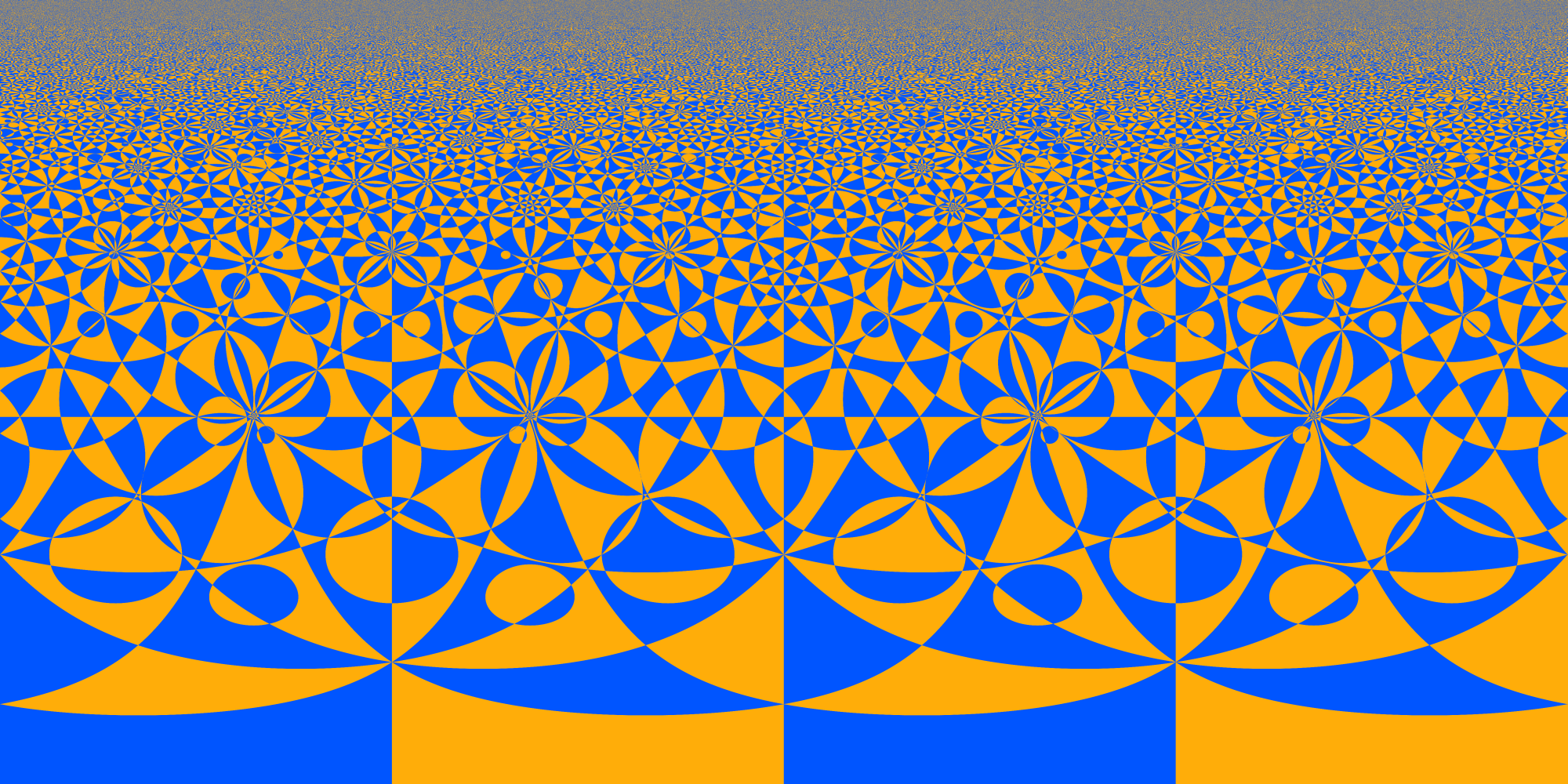# Inversive Geometry

• Convenient container to work with 3 dimensional hyperbolic tilings is 3D Inversive (or Moebius) Geometry. It can contain upper half space model and ball model of `H^4`.
• Geometric transformations are reflections in planes and inversions in spheres (splanes) and arbitrary compositions.
• Each splane divide space into two sets interior and exterior. Specific orientation is assigned to splanes by direction of normal that points to the exterior of the splane.
• Regions are defined as intersection of interiors of splanes.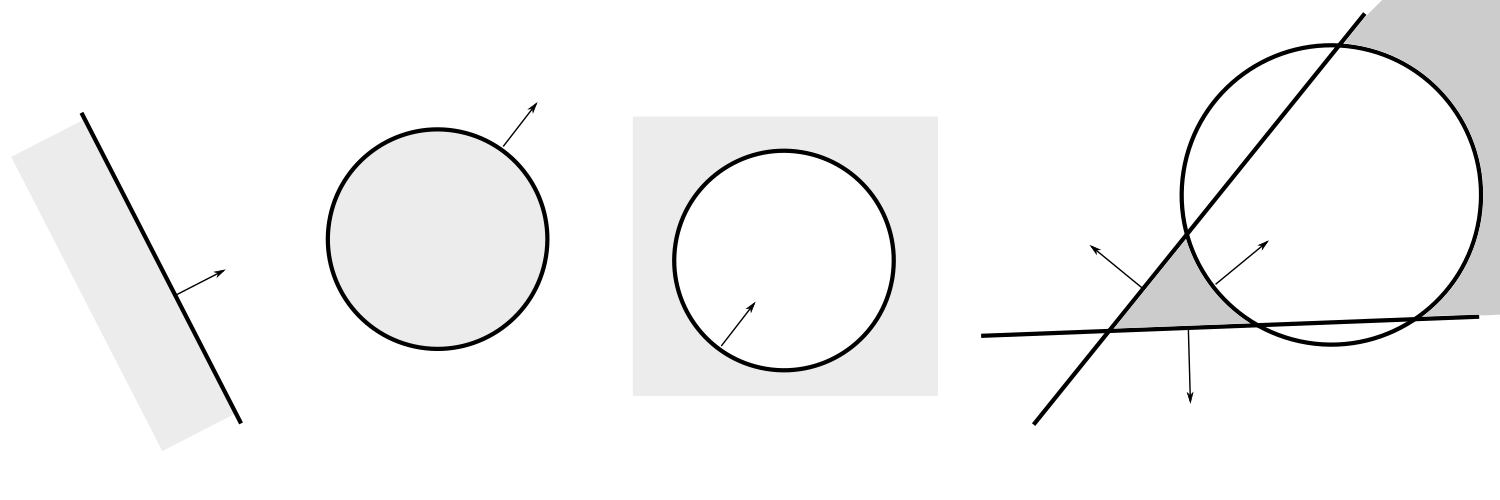Region on the right looks like two components region, however it is an infinite cheese wedge with a spherical hole.

# Reflection in planesPlane `P {N, d}` is defined via `N` - vector of external normal and `d` - distance of plane to origin `O`.

Reflection in plane `P` maps point `A` into point `P(A)`
`P(A) = A - 2 << A - d N, N >> N`.
Where `<< , >>` is scalar product.

Signed distance of point `A` to to plane `P` is
`P(S, A) = << A, N >> - d`

Here we have mapped interior region of the plane (on the right) on the exterior region on the left.

# Inversion in spheres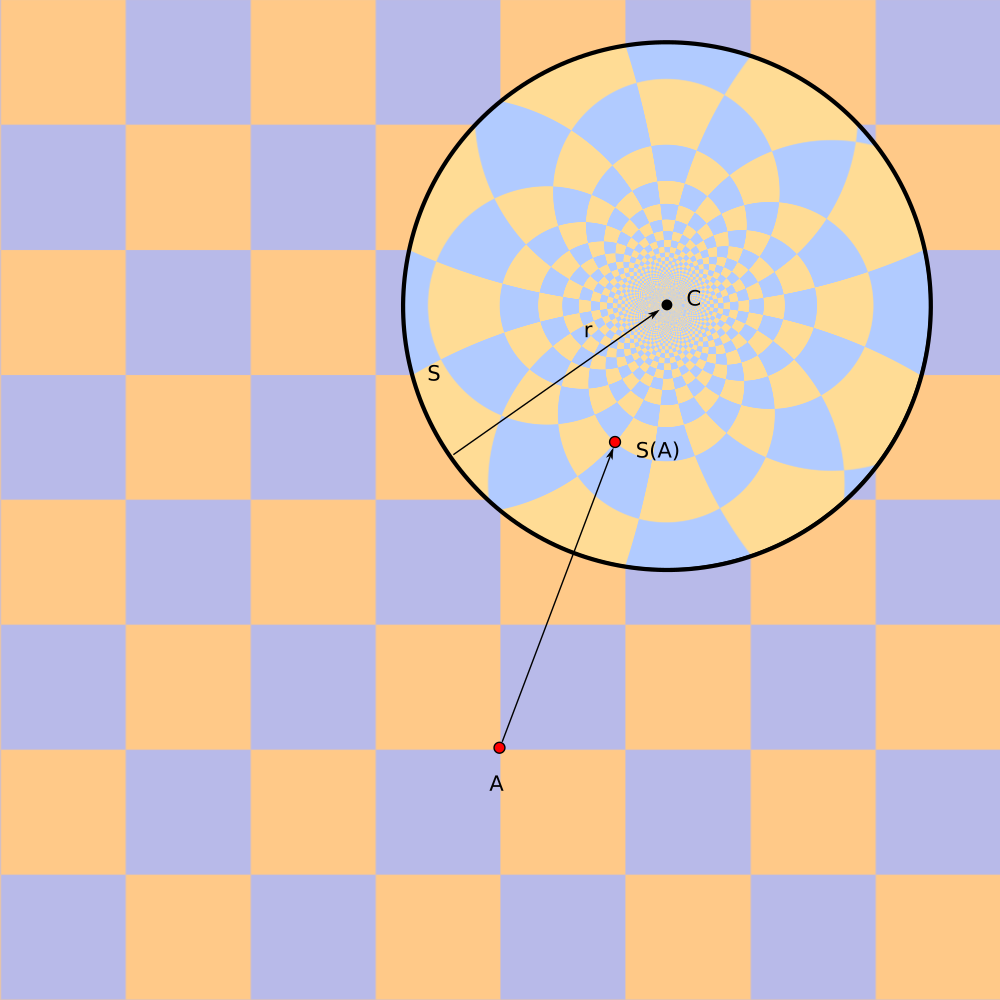Sphere `S{C,r}` is defined via center `C` and signed radius `r`.

Positive radius means interior of splane `S` is region inside of the ball.

Negative radius means interior of splane is region outside of the ball.

Inversion in sphere `S` maps point `A` into point `S(A)`
`S(A) = C + r^2/|A - C|^2 (A-C)`

Signed distance of point `A` to to sphere `S` is
`D(S, A) = sign(r) (|A-C| - |r|)`

# Planes are spheresInversion is sphere becomes reflection in plane when radius of sphere tends to infinity.

# Planes are spheres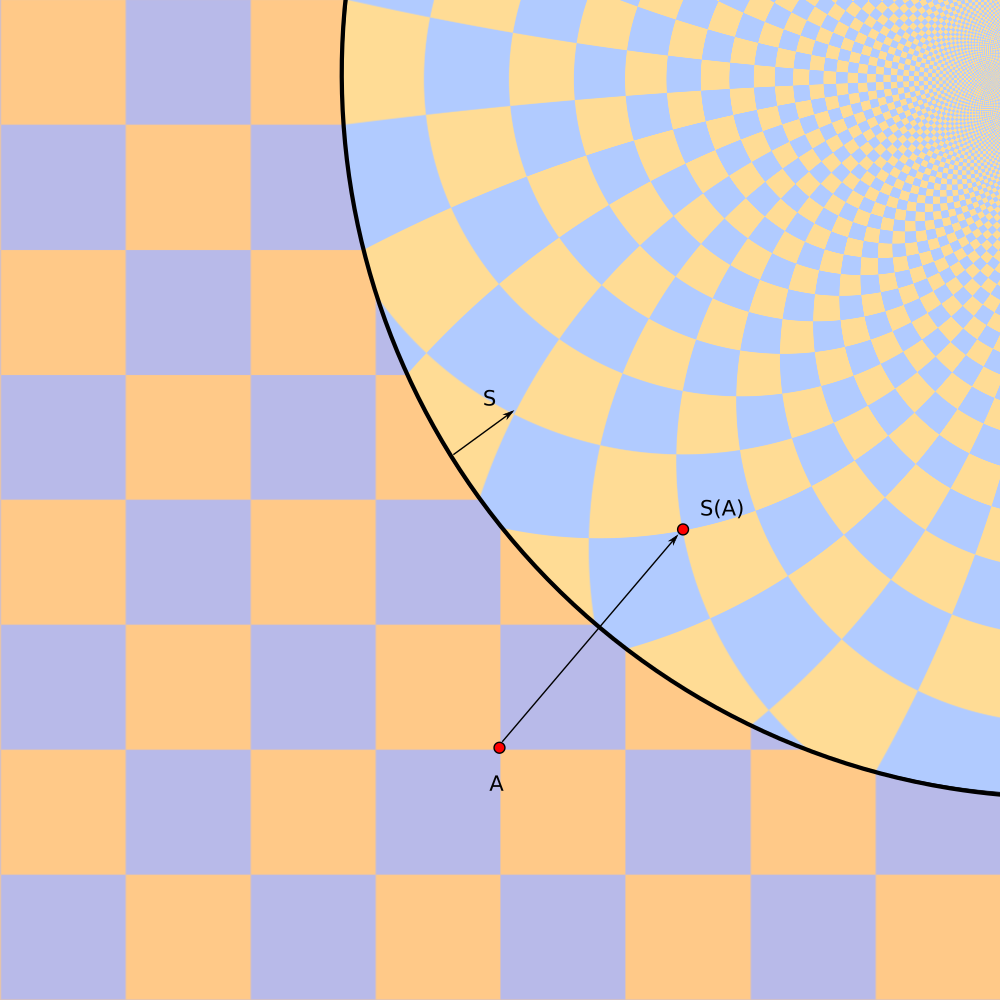Inversion is sphere becomes reflection in plane when radius of sphere tends to infinity.

# Planes are spheres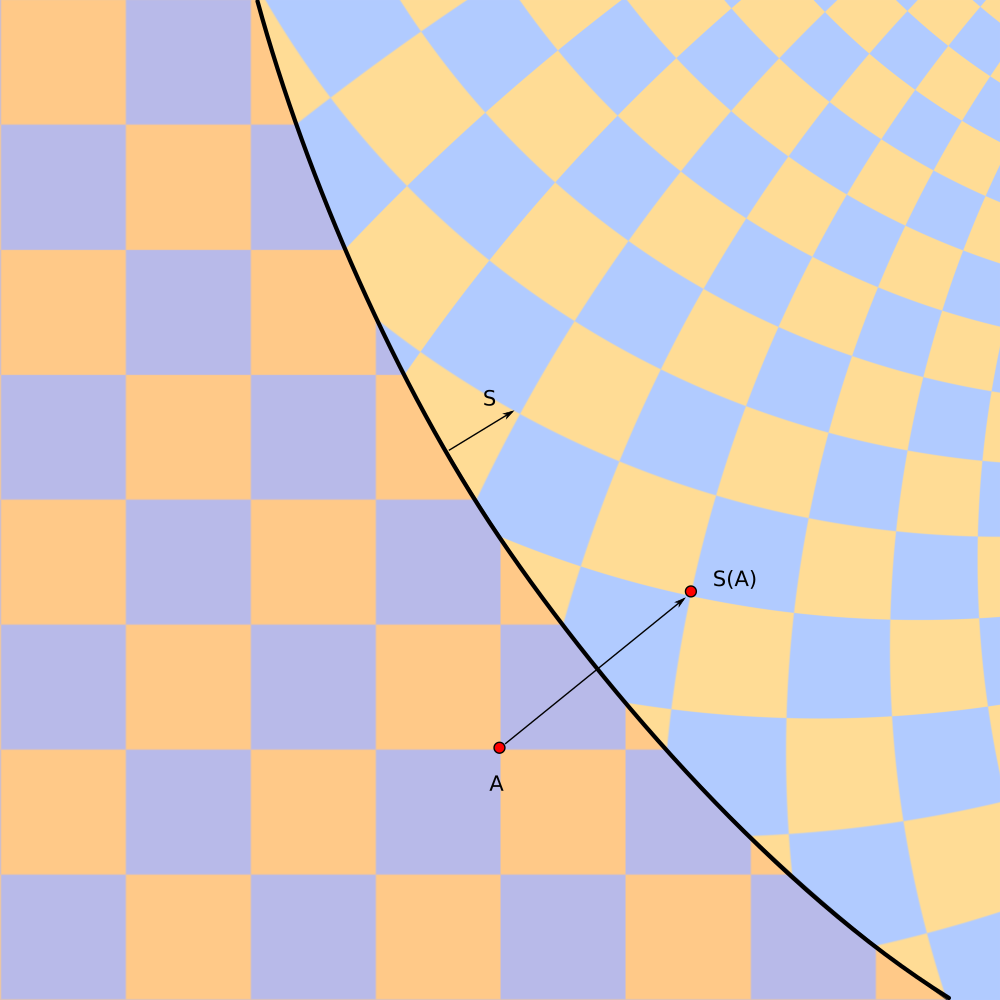Inversion is sphere becomes reflection in plane when radius of sphere tends to infinity.

# Planes are spheres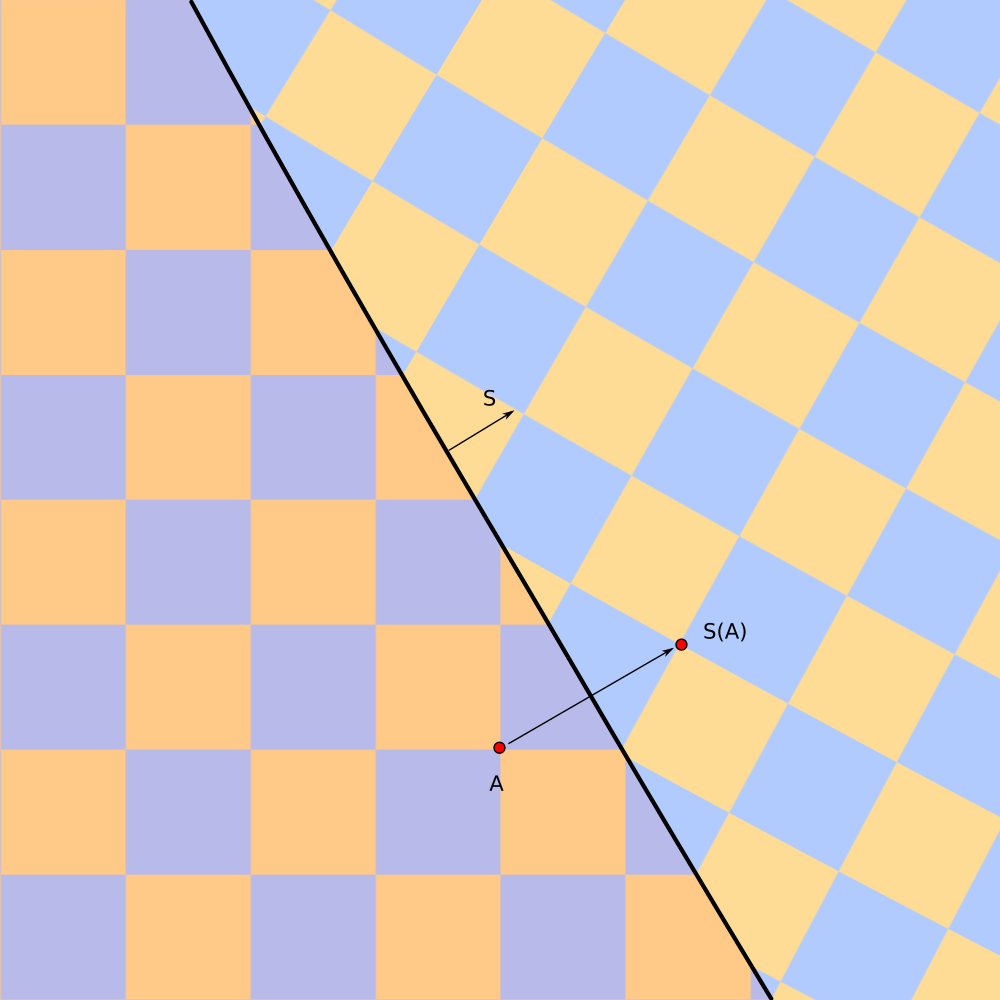Inversion is sphere becomes reflection in plane when radius of sphere tends to infinity.

# How to construct a tiling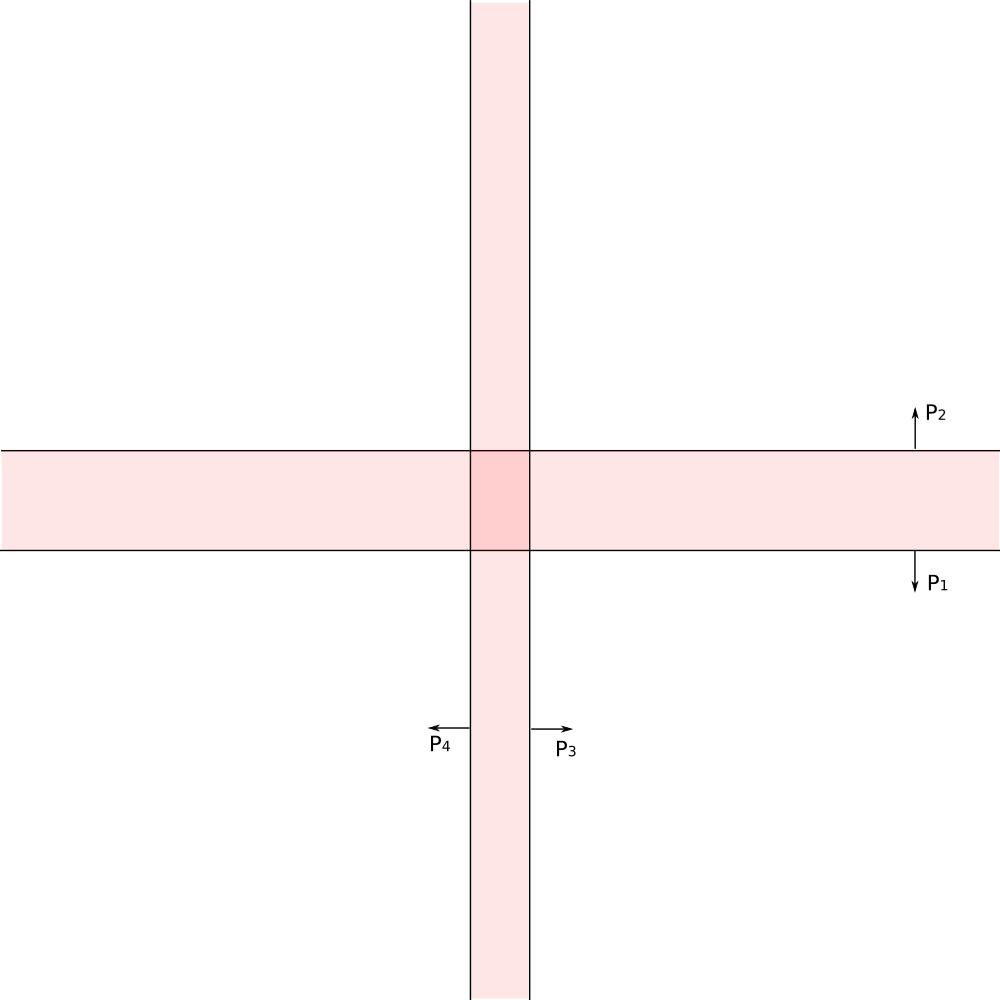Tiling can be constructed in several steps

• Build Fundamental Domain - region bounded by finite number of splanes (`P_1`, `P_2`, `P_3`, `P_3`)
• Dihedral angles between splanes are integral fraction of `pi`: `a_(ij) = pi/m_(ij)`
• Build symmetry group generated by reflections in splanes

# How to construct a tiling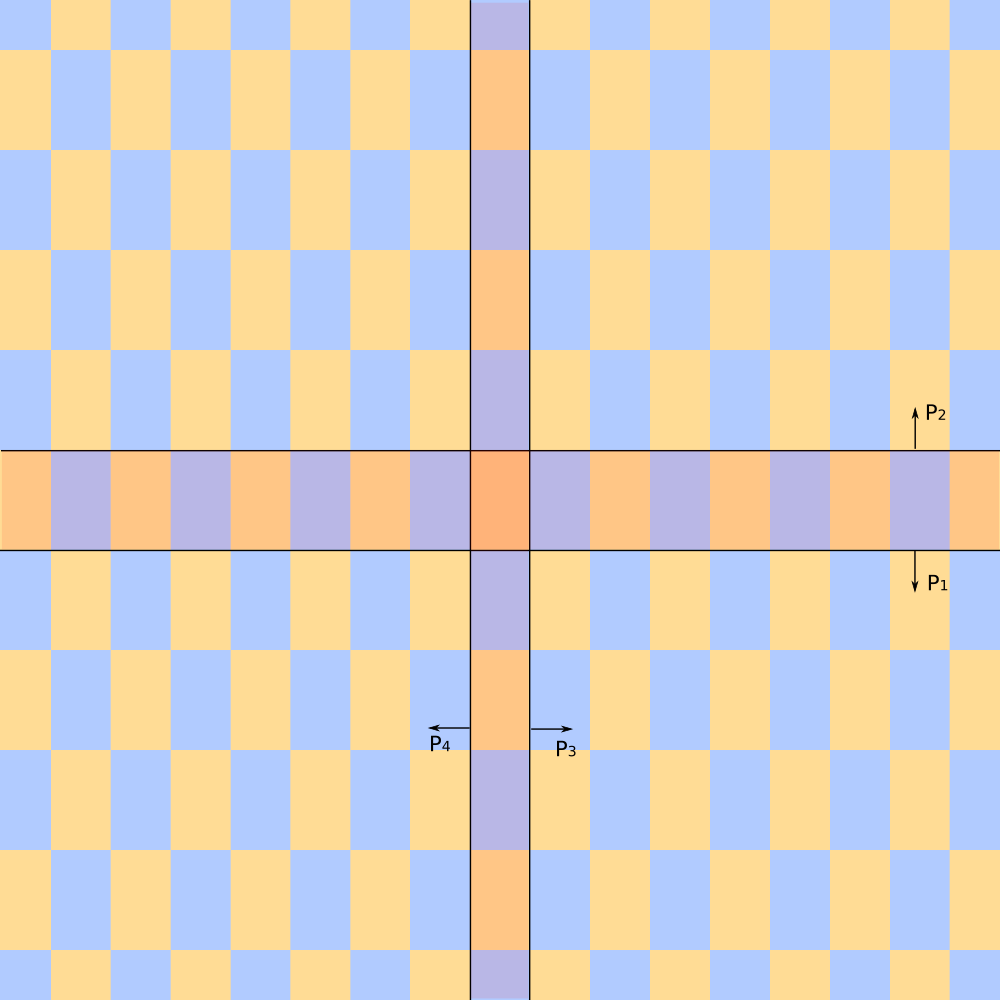Tiling can be constructed in several steps

• Build Fundamental Domain - region bounded by finite number of splanes (`P_1`, `P_2`, `P_3`, `P_3`)
• Dihedral angles between splanes are integral fraction of `pi`: `a_(ij) = pi/m_(ij)`
• Build symmetry group generated by reflections in splanes
• Fill space by copies of Fundamental Domain transformed by all transformations of the group.

# How to draw the tiling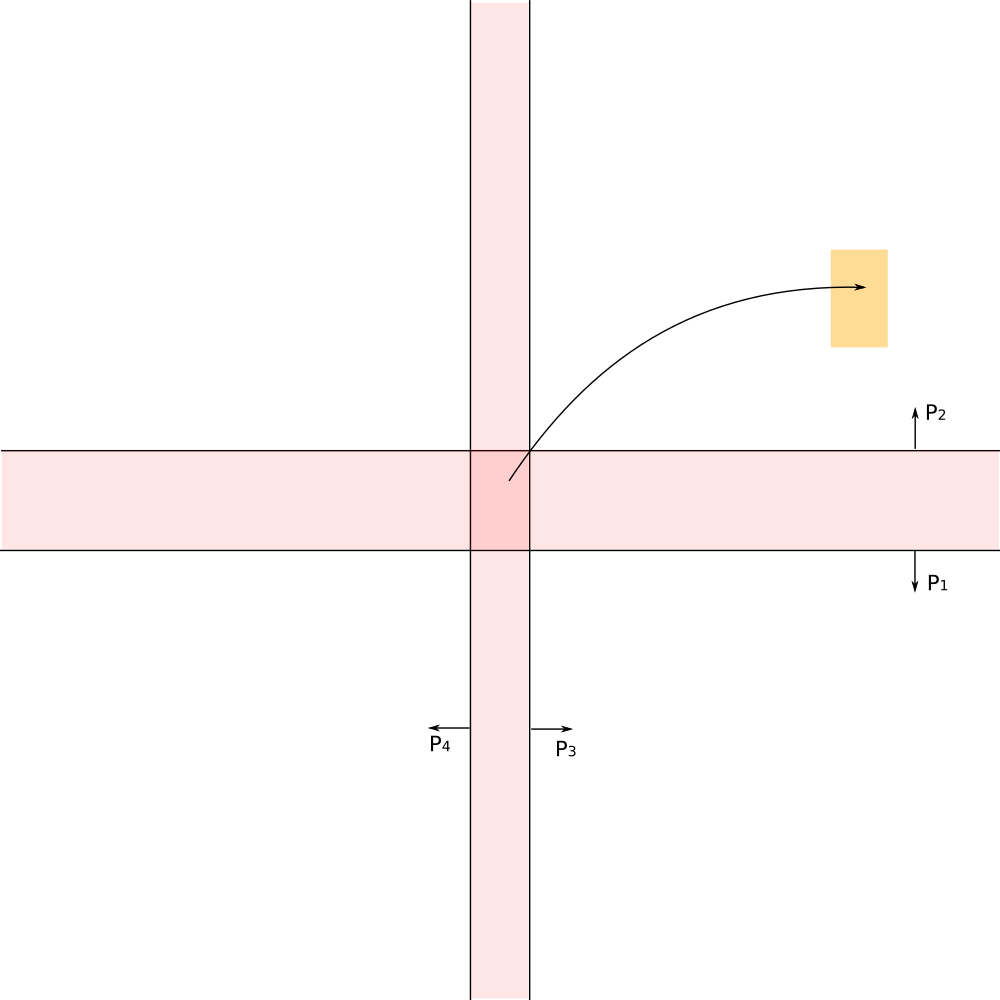Straightforward appoach - apply each of transformation of the group to the fundamental domain.

Troubles

• Groups are usually infinite
• Fundamental Domain often is infinite
• Transformations of the group may act on the fundamental domain in rather complex way, mapping finite regions into infinity.

# How to draw the tiling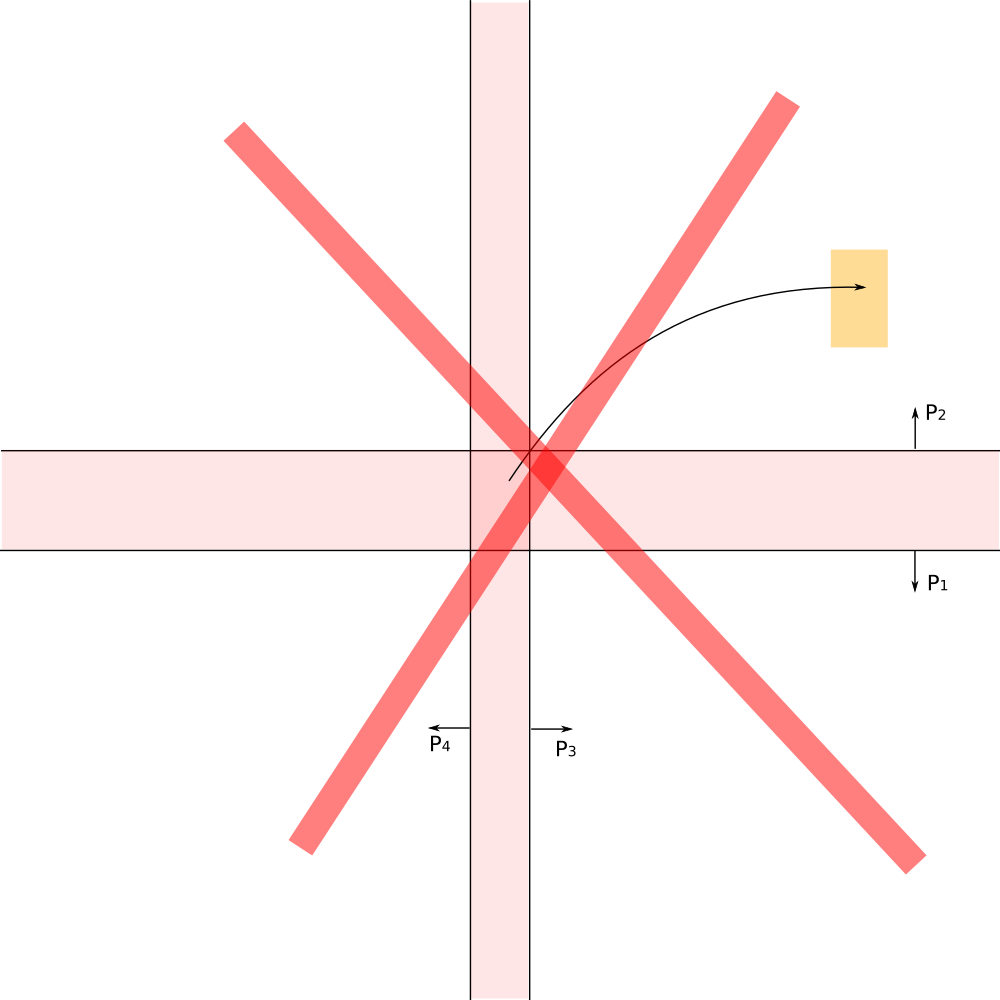Straightforward appoach - apply each of transformation of the group to the fundamental domain.

Troubles

• Groups are usually infinite
• Fundamental Domain often is infinite
• Transformations of the group may act on the fundamental domain in rather complex way, mapping finite regions into infinity.

Different apporach is needed!

# Reverse Pixels Mapping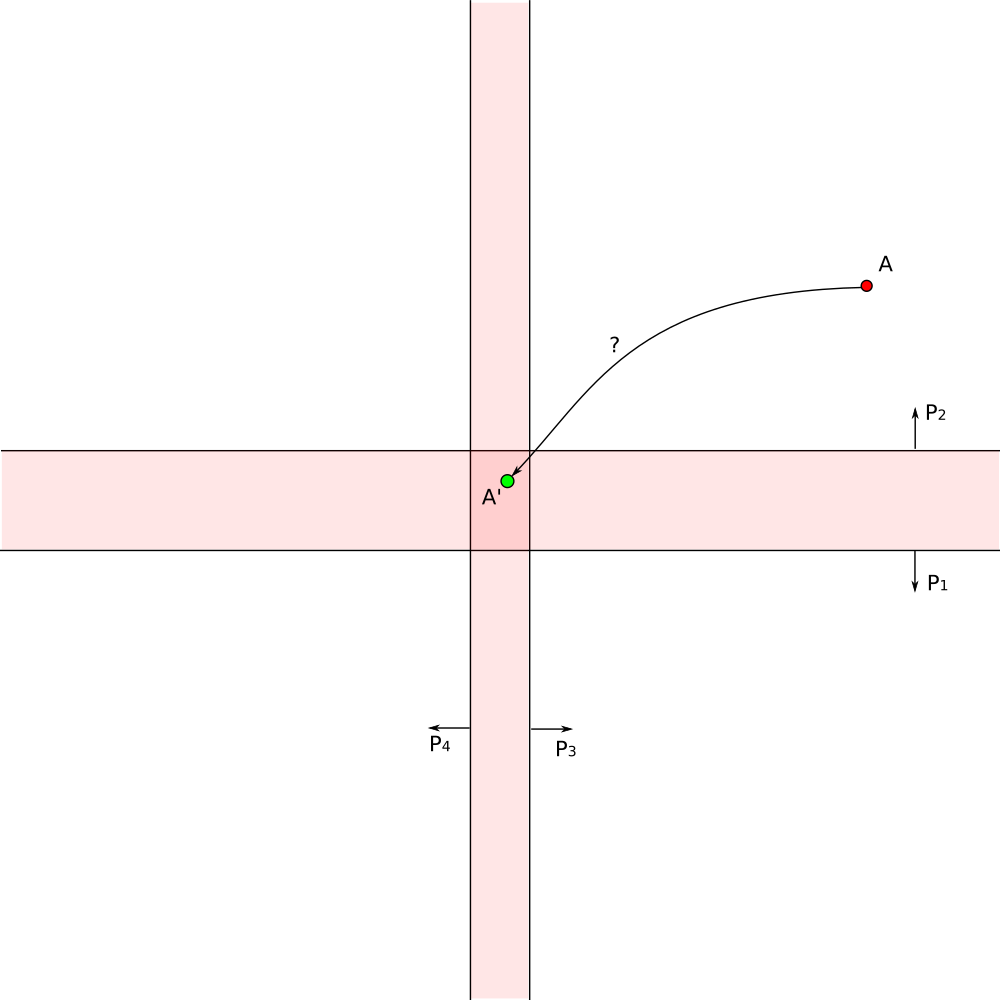Different approach - draw only pixels which we can see.

For each visible pixel on the screen find transformation which maps it to the corresponding pixel in the fundamental domain.

Color the pixel with color of the corresponding pixel in the fundamentral domain.

# Search of Reverse Mapping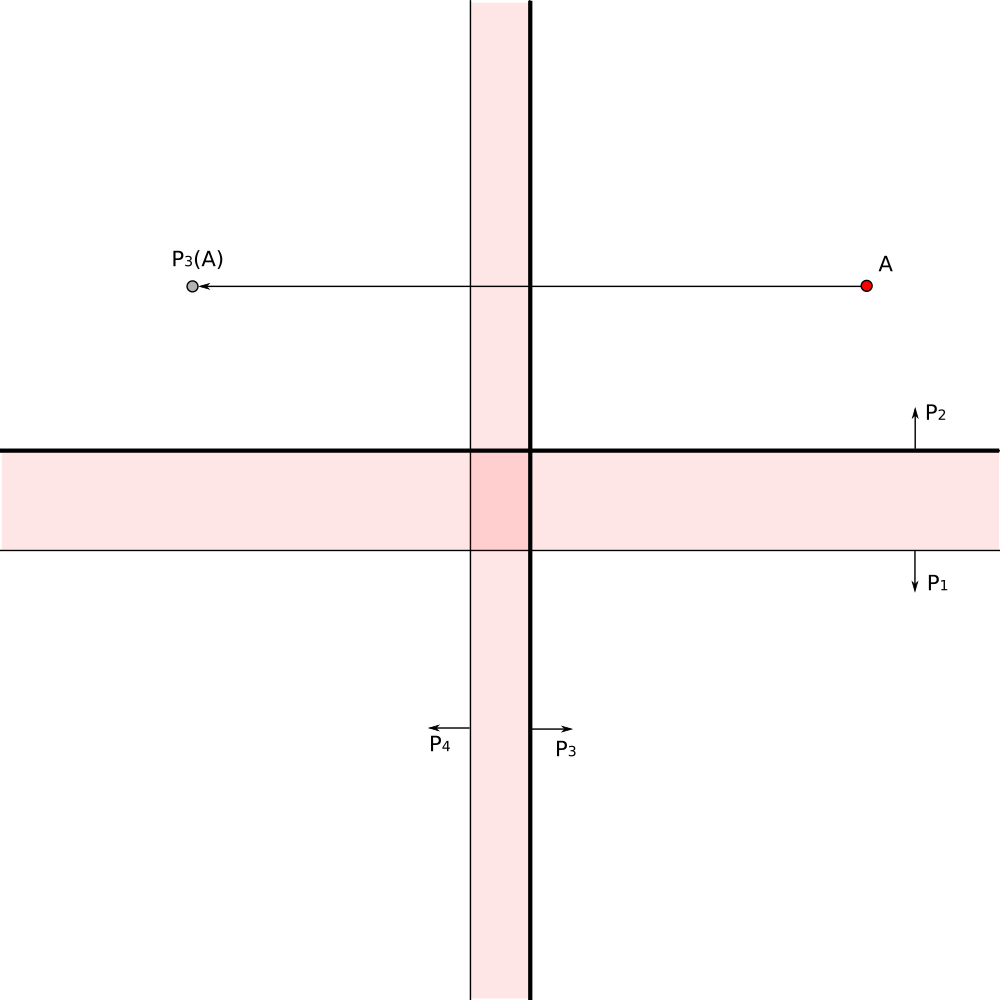• Check the point location relative to each generator.
• Reflect point in first found generator which has point in its exterior

# Search of Reverse Mapping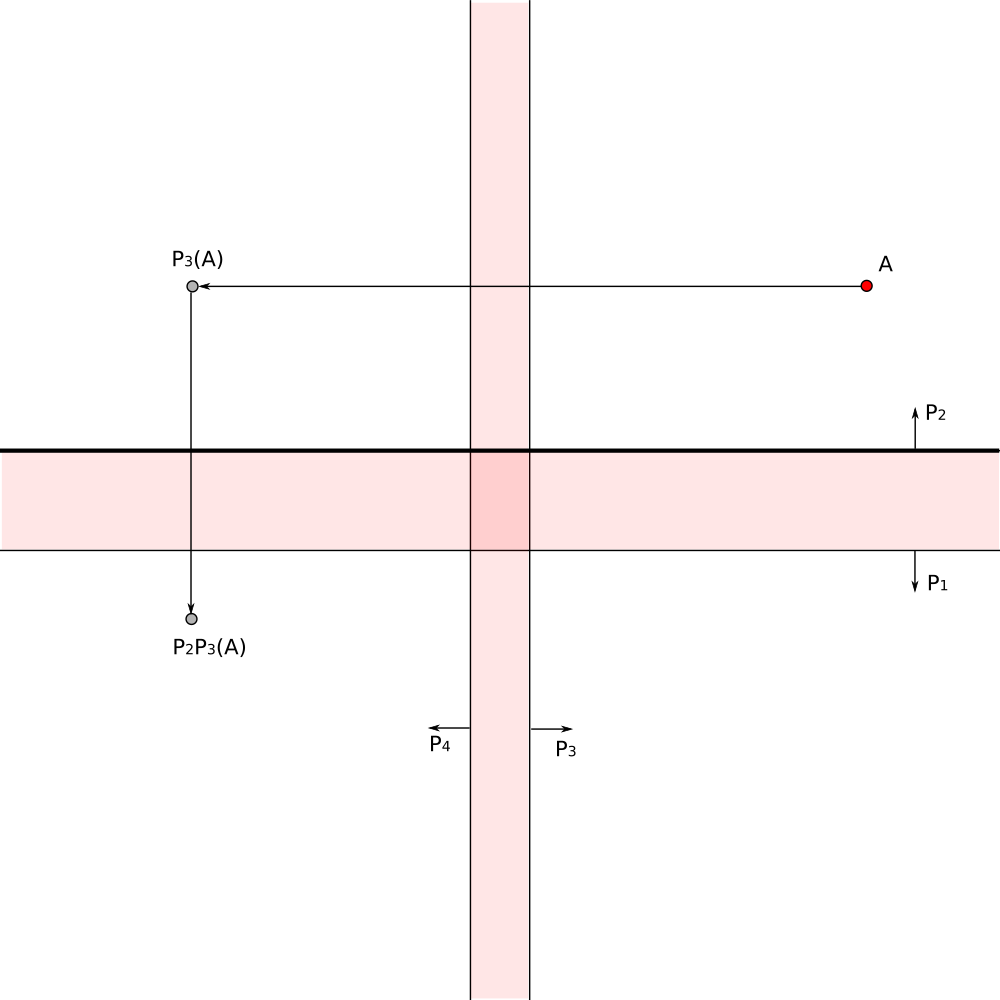• Check the point location relative to each generator.
• Reflect point in first found generator which has point in its exterior
• Continue the process

# Search of Reverse Mapping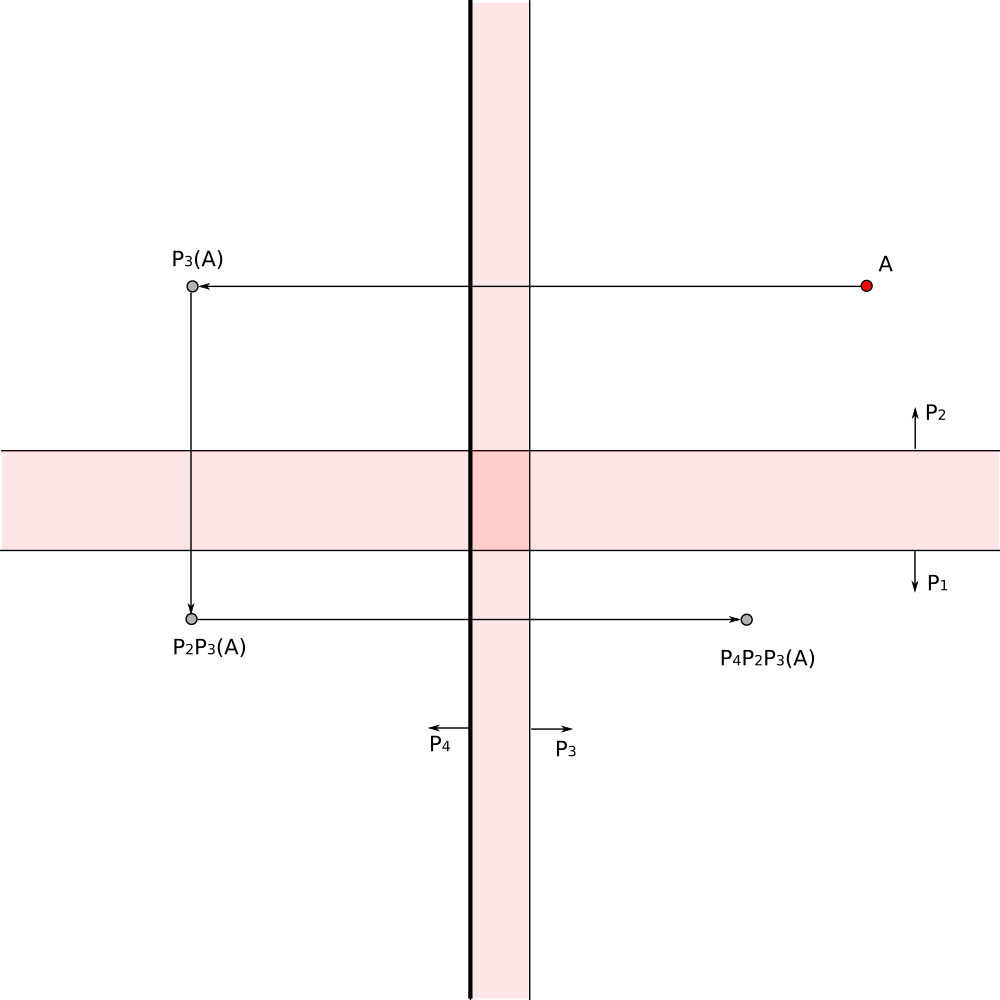• Check the point location relative to each generator.
• Reflect point in first found generator which has point in its exterior
• Continue the process

# Search of Reverse Mapping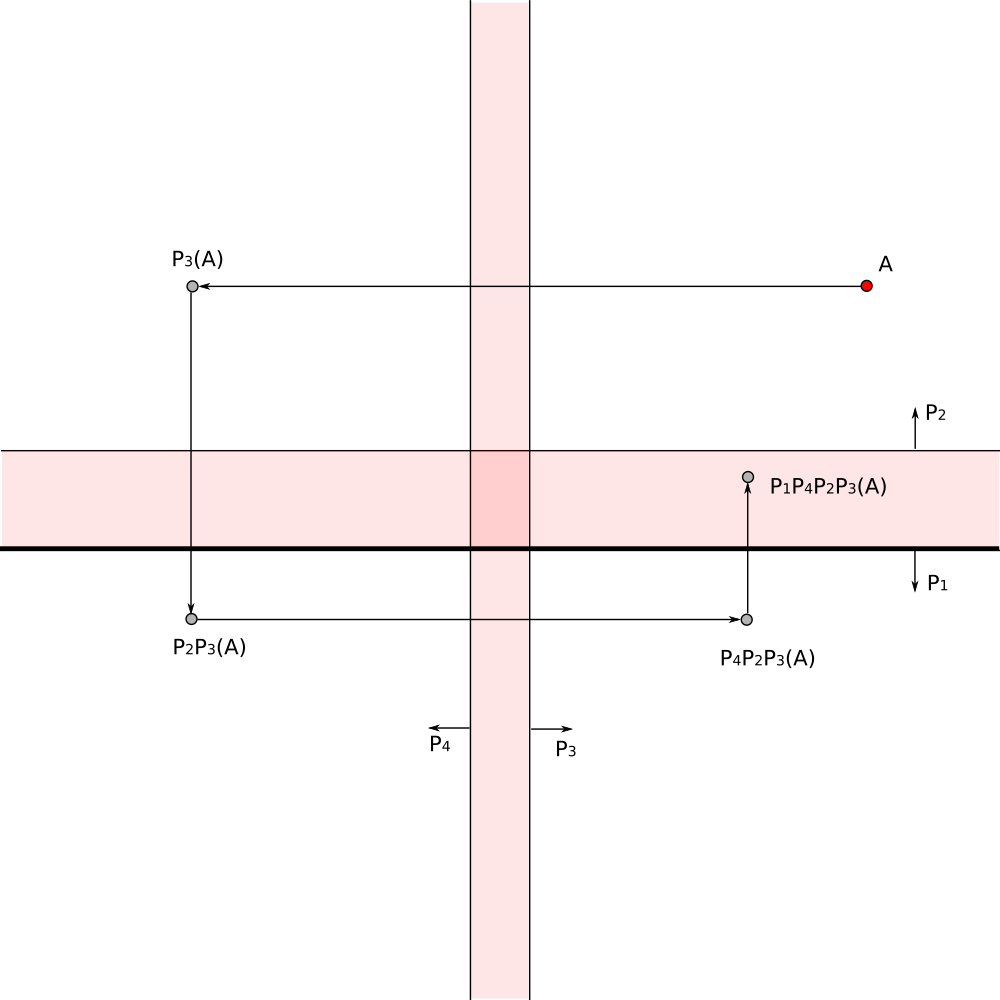• Check the point location relative to each generator.
• Reflect point in first found generator which has point in its exterior
• Continue the process

# Search of Reverse Mapping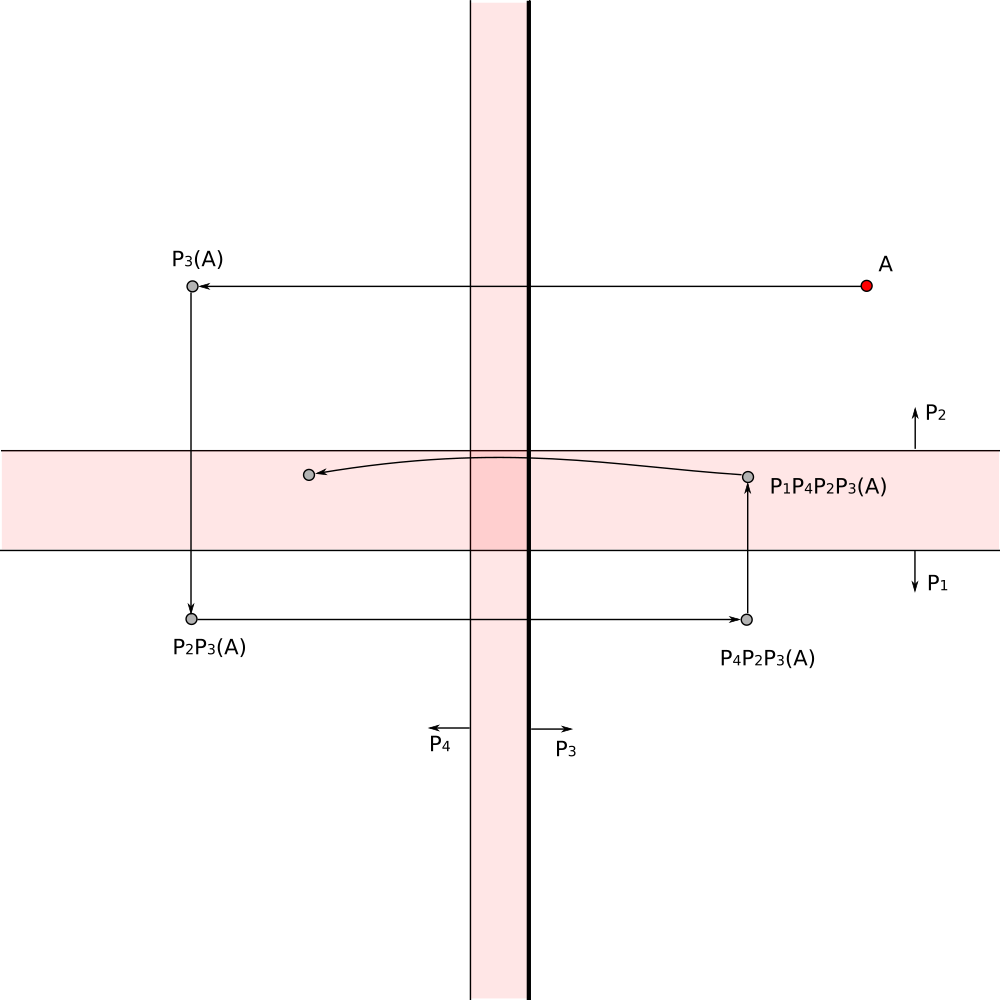• Check the point location relative to each generator.
• Reflect point in first found generator which has point in its exterior
• Continue the process

# Search of Reverse Mapping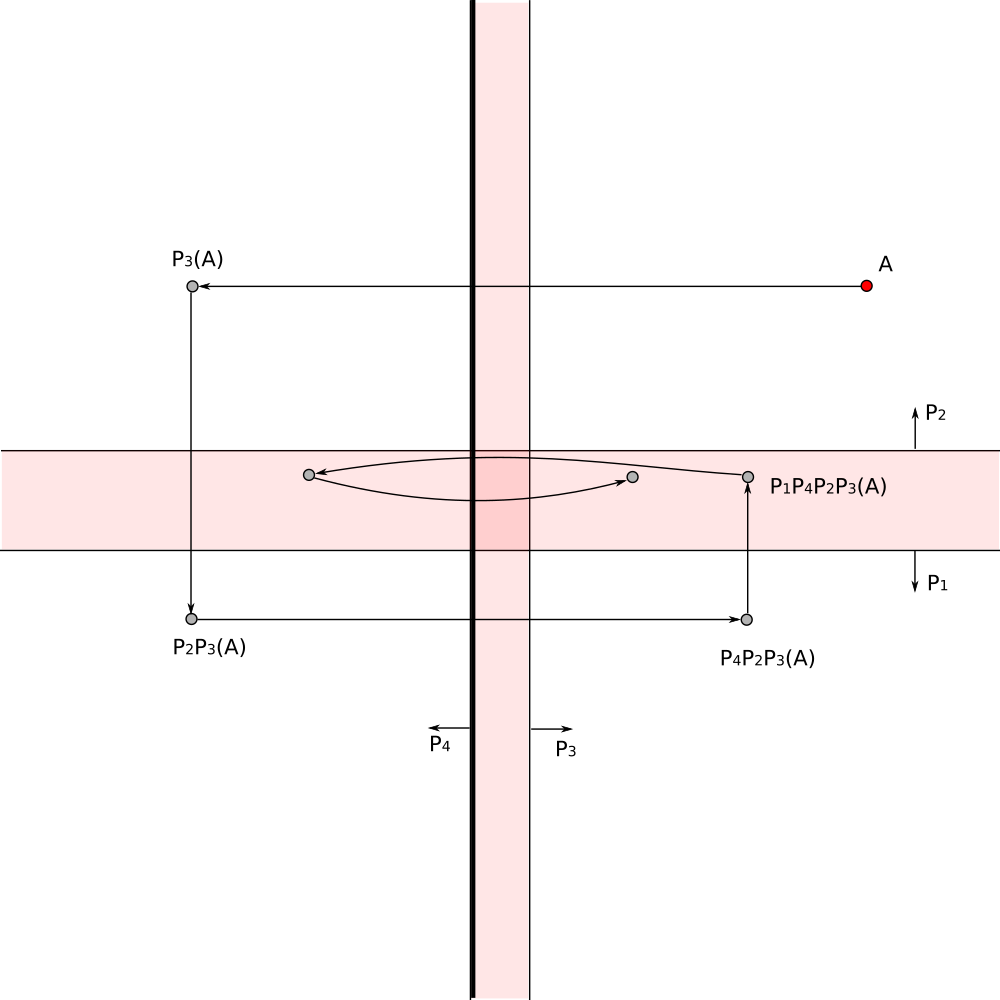• Check the point location relative to each generator.
• Reflect point in first found generator which has point in its exterior
• Continue the process

# Search of Reverse Mapping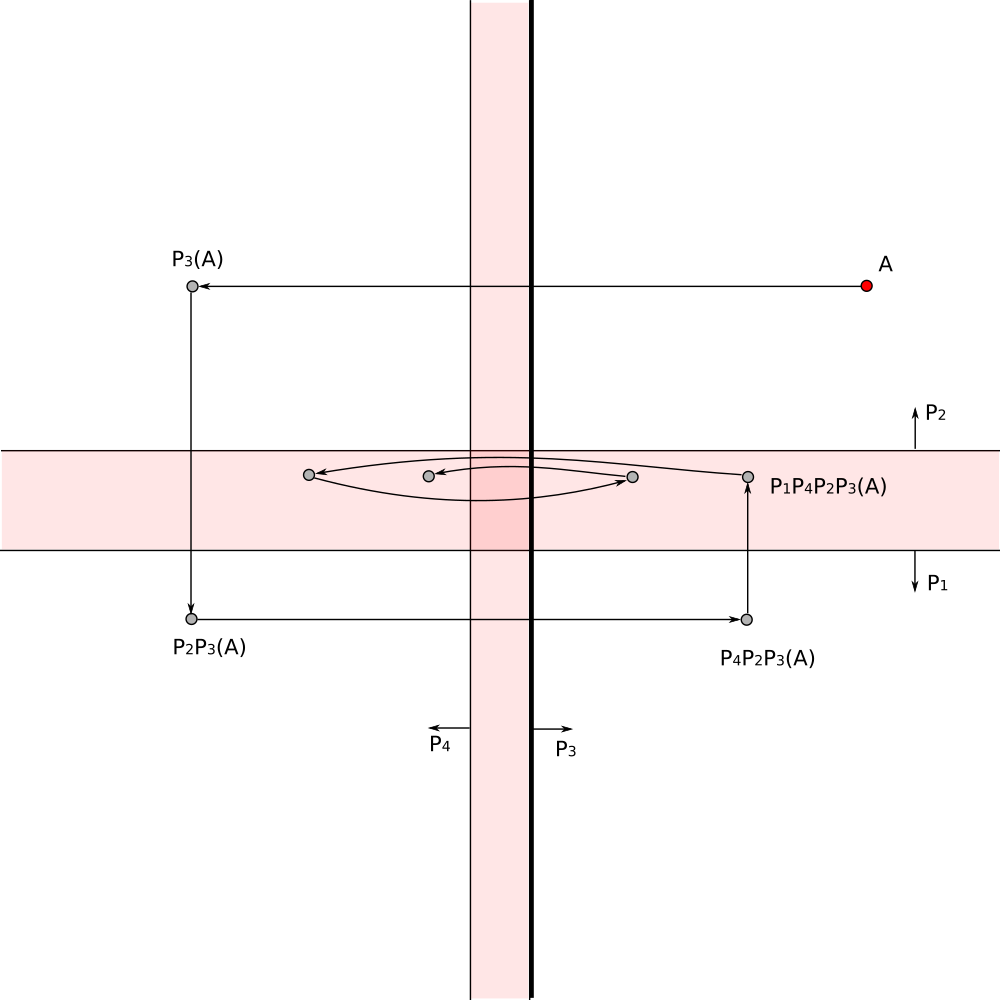• Check the point location relative to each generator.
• Reflect point in first found generator which has point in its exterior
• Continue the process

# Search of Reverse Mapping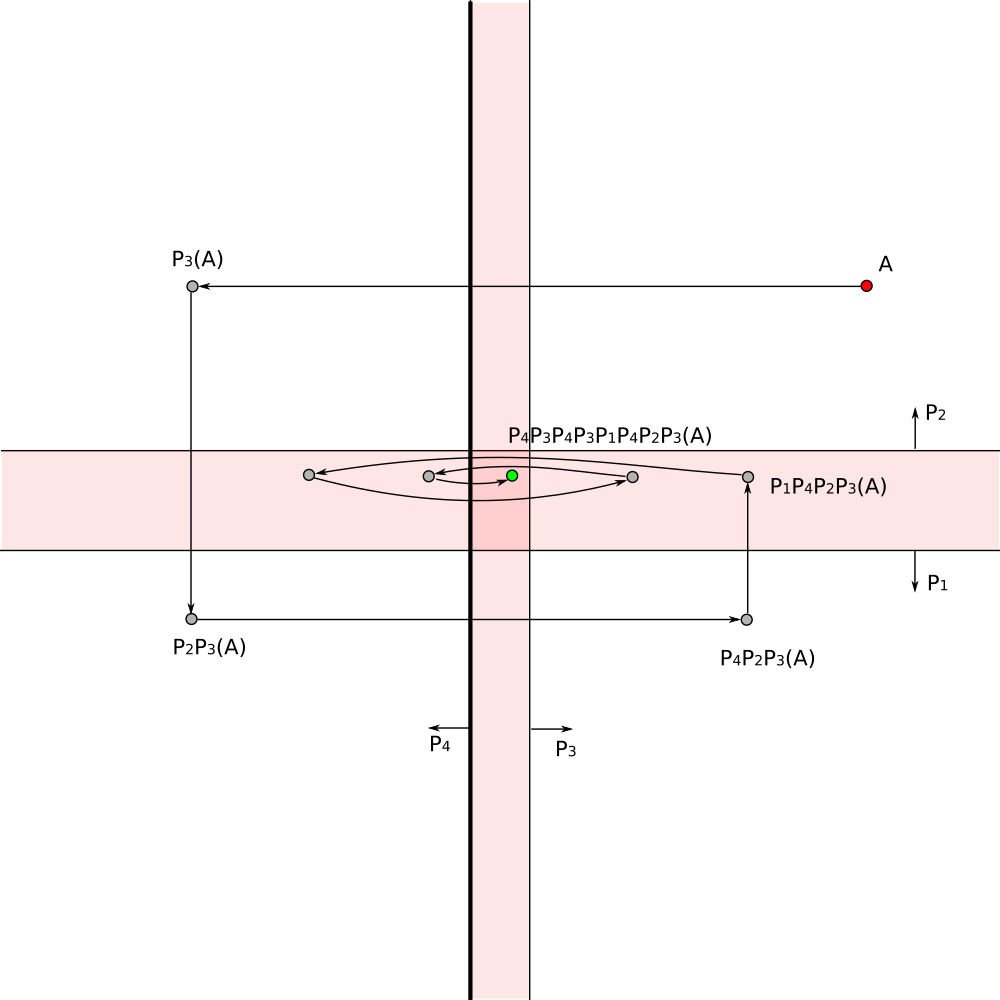• Check the point location relative to each generator.
• Reflect point in first found generator which has point in its exterior
• Continue the process until the point is inside of each generator.

# Search of Reverse Mapping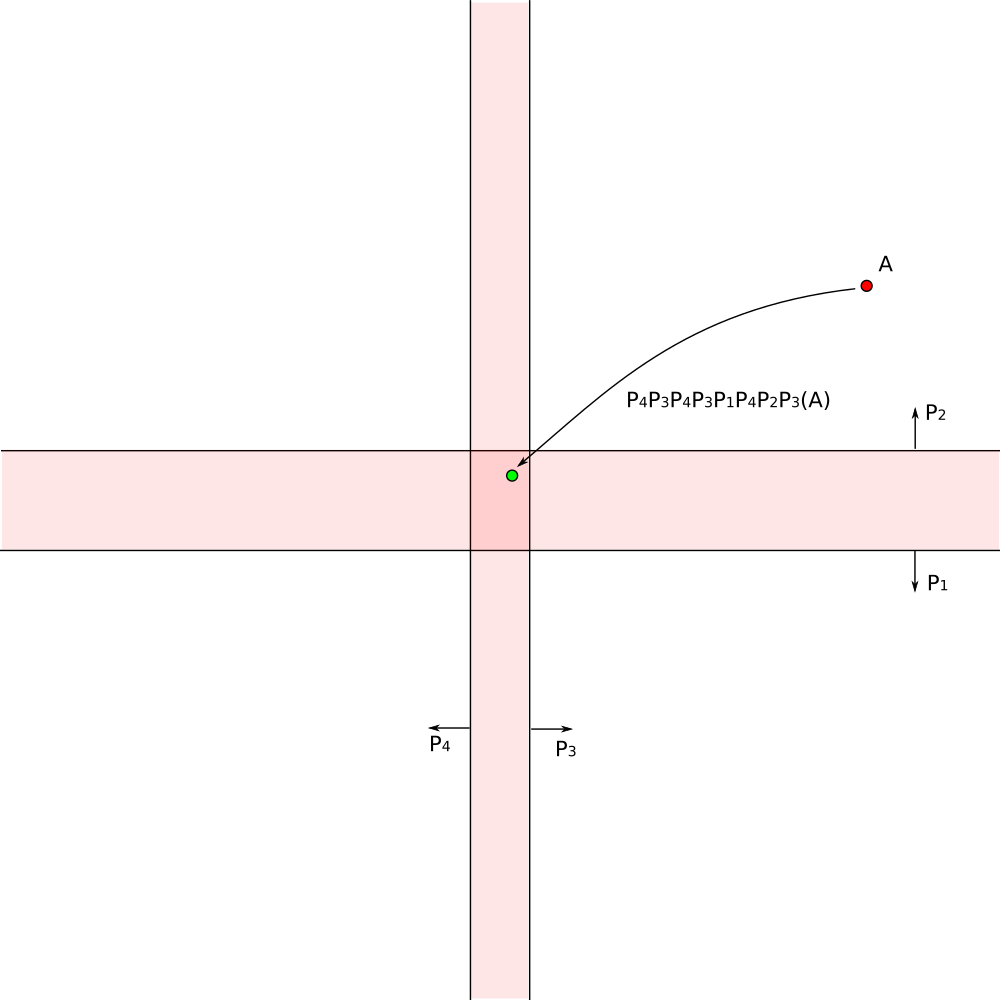The composition of reflections gives the required transformation

# Coloring the Pixels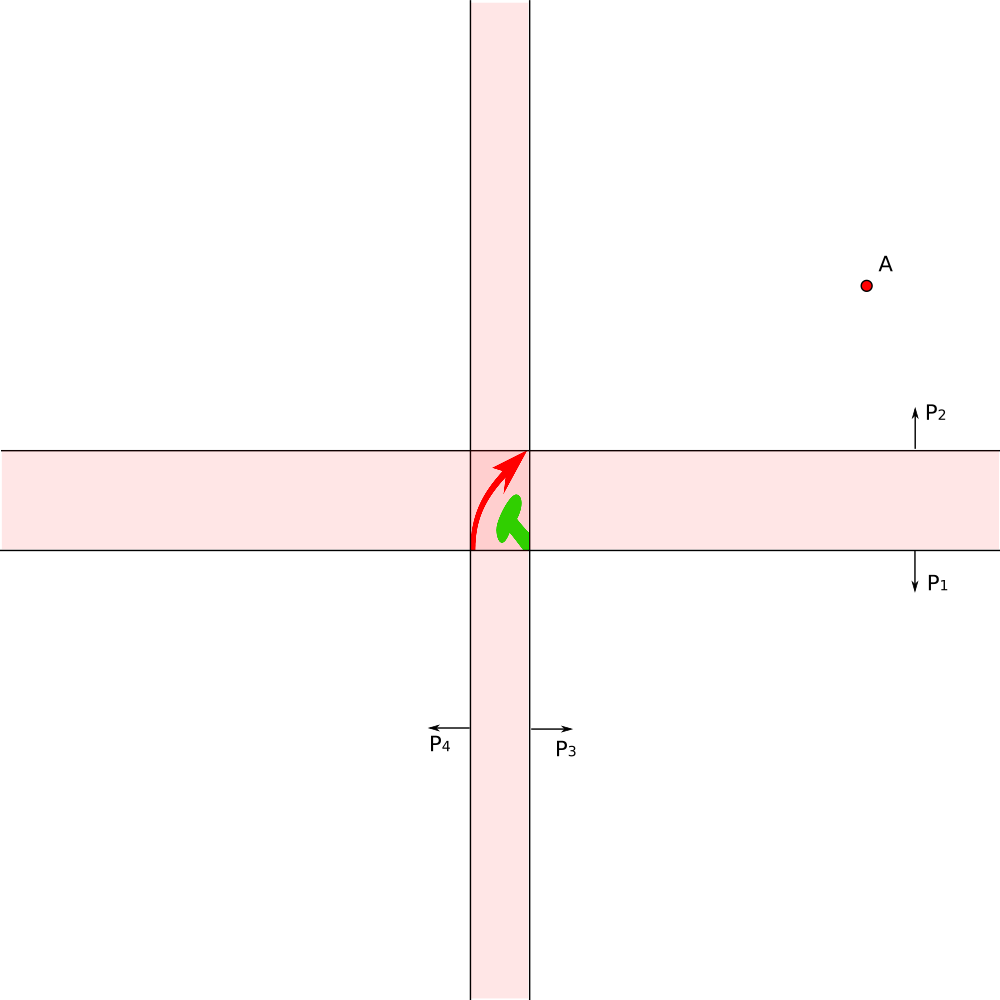Arbitrary pattern can be placed inside of the fundamental domain

# Coloring the Pixels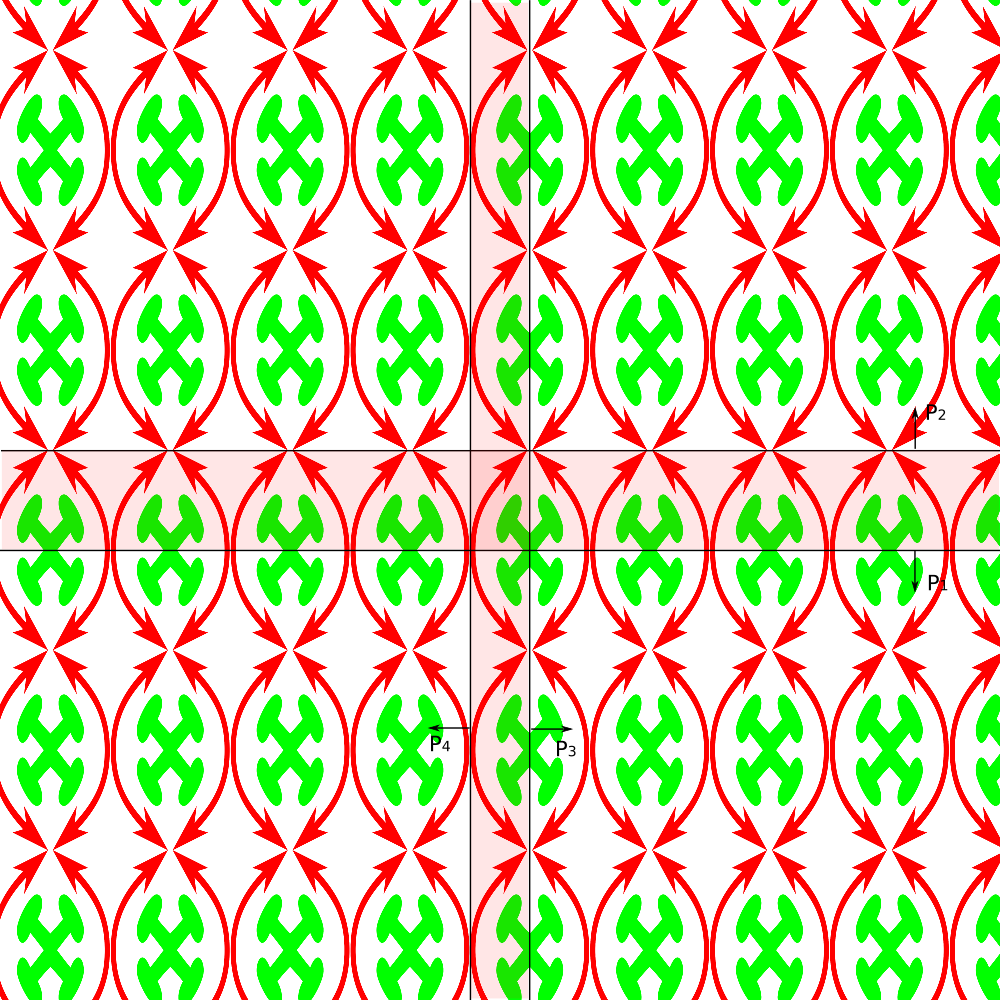Arbitrary pattern can be placed inside of the fundamental domain

The pattern is reflected to the whole visible space

The calculations for each pixel are independent and can be run on GPU in paralel in real time

# Coset coloringAnother way to color the pixel is coset coloring.

Here pixels are assigned different color according to coset of the orientation preserving subgroup of index 2.

In this simplest case coset coloring can be obtained by counting number of reflections needed to map pixel to fundamental domain and assigning one color if count is even and another color if count is odd.

# Coset coloring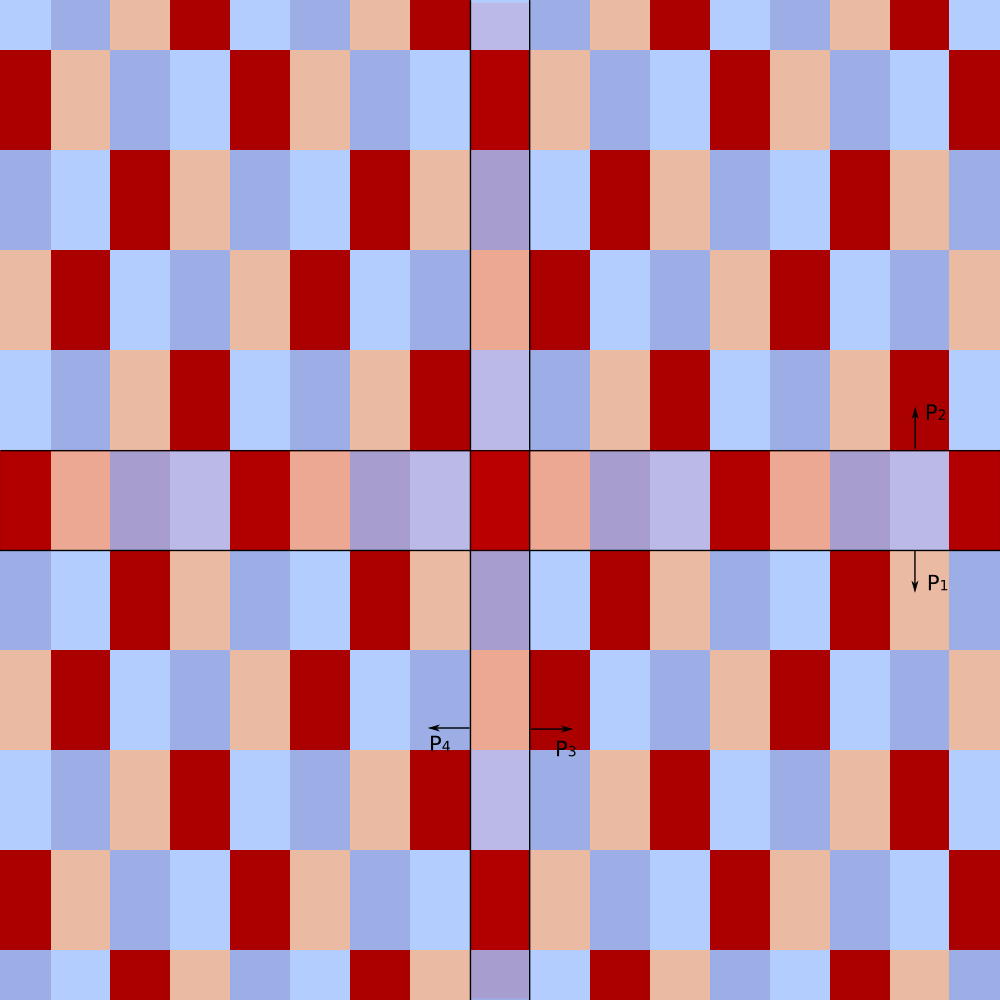Color count equals to the index of subgroup.

# Coset coloringColor count equals to the index of subgroup.

The index of subgroup of infinite group can be arbitrary large.

This is coset coloring with 10 colors

# Building Hyperbolic Tiling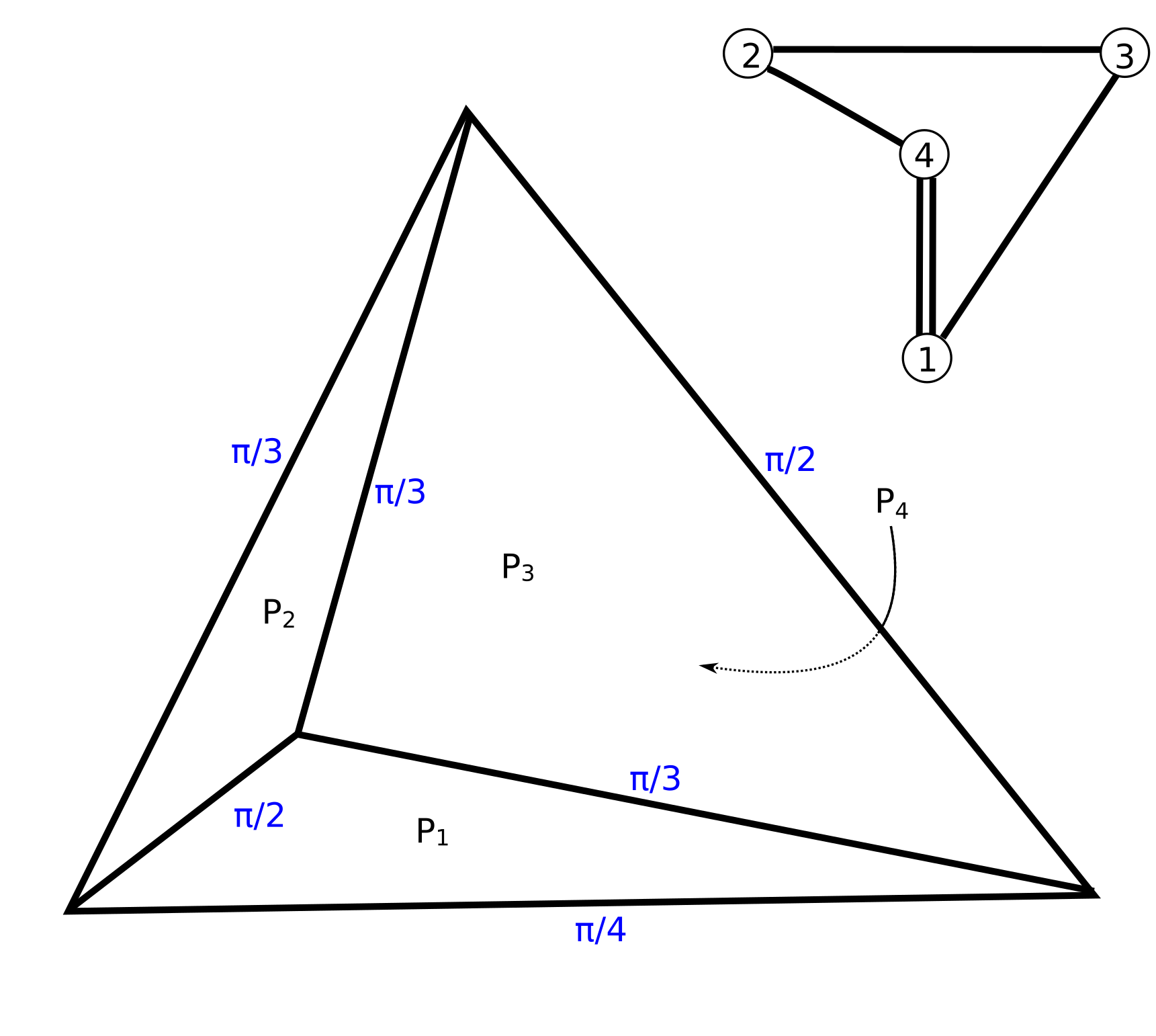Simplest hyperbolic tilings are tiling with tetrahedra.

Hyperbolic tetrahedron with all finite vertices.

It is convenient to display tetrahedra using Coxeter diagram.

Each plane is shown as small circle.

For each pair of faces `(i,j)` with dihedral angle `alpha = pi/m_(ij)` the corresponding pair of circles `i,j` is connected with `(m_(ij)-2)` lines.

# Building Tetrahedron 1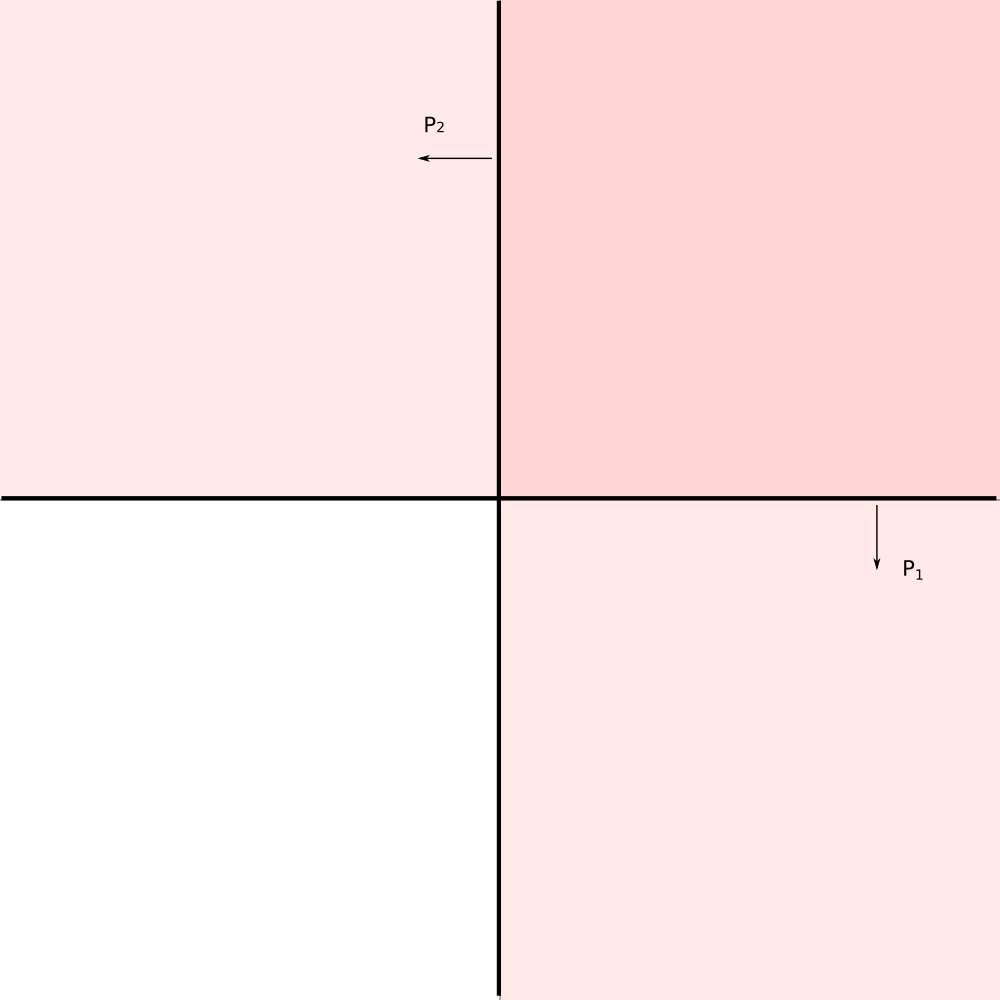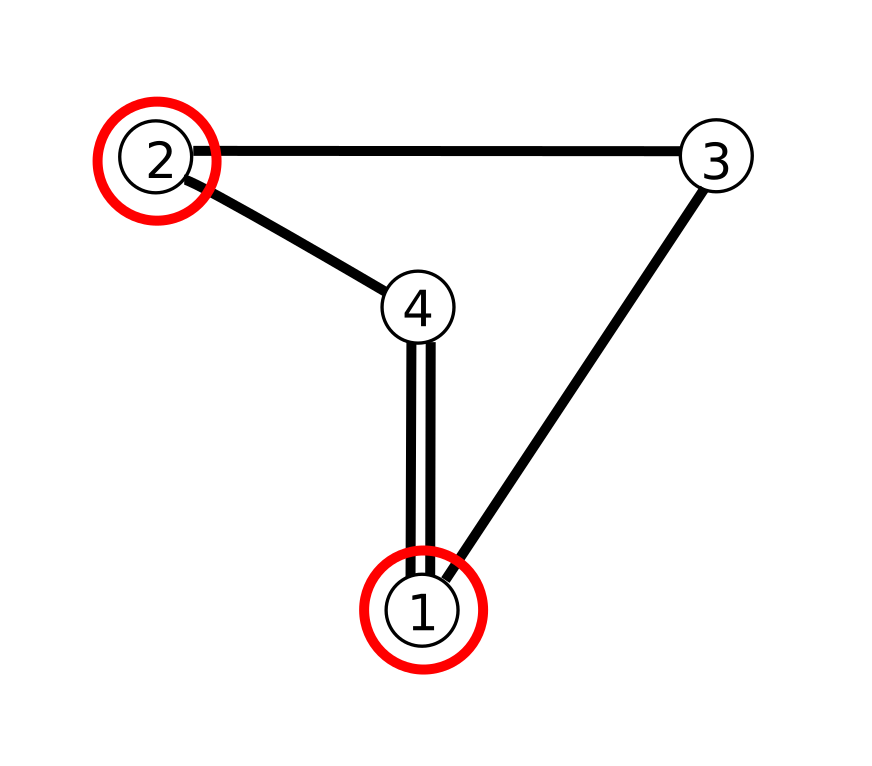-->

Let's work in the upper half space model of `H^3`. Horizon of `H^3` lies in the xy-plane `(z = 0)`

Lets place planes `P_1` and `P_2` orthogonal to Z axis and orthogonal to each other.

# Building Tetrahedron 2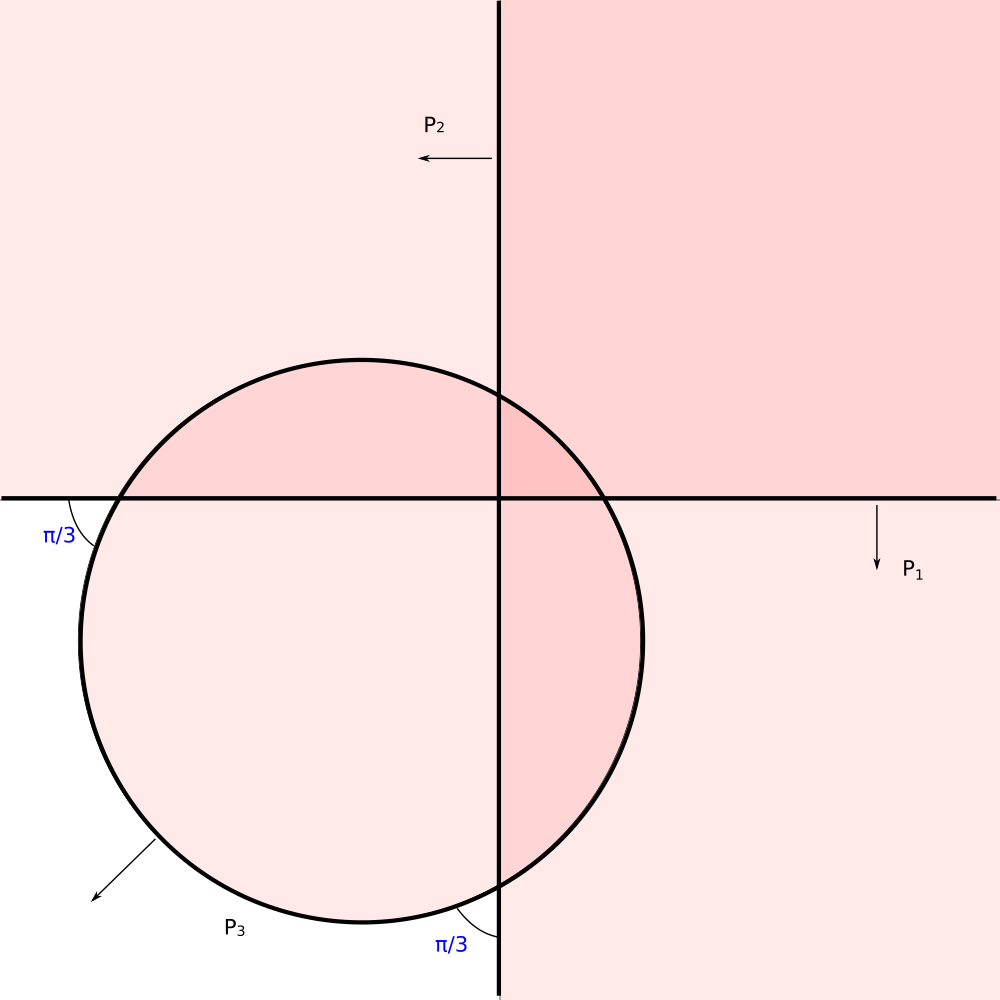Plane `P_3` is a sphere centered at the horizon.

Dihedral angles of its intersections with planes `P_1` and `P_2` are `alpha_(13) = pi/3` and `alpha_(13) = pi/3`.

# Building Tetrahedron 3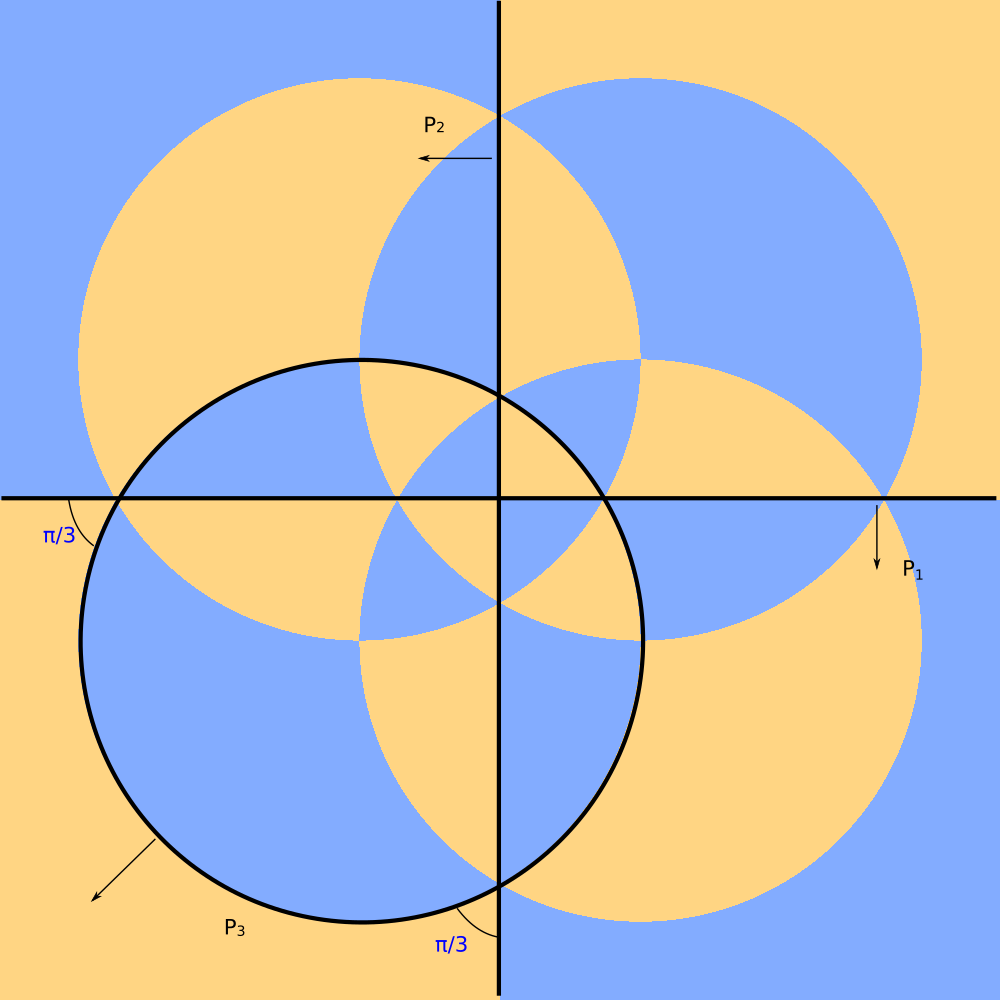Tiling generated by splanes `P_1` `P_2` `P_3`.

It is stereographic projection of the spherical tiling `**332`.

# Building Tetrahedron 4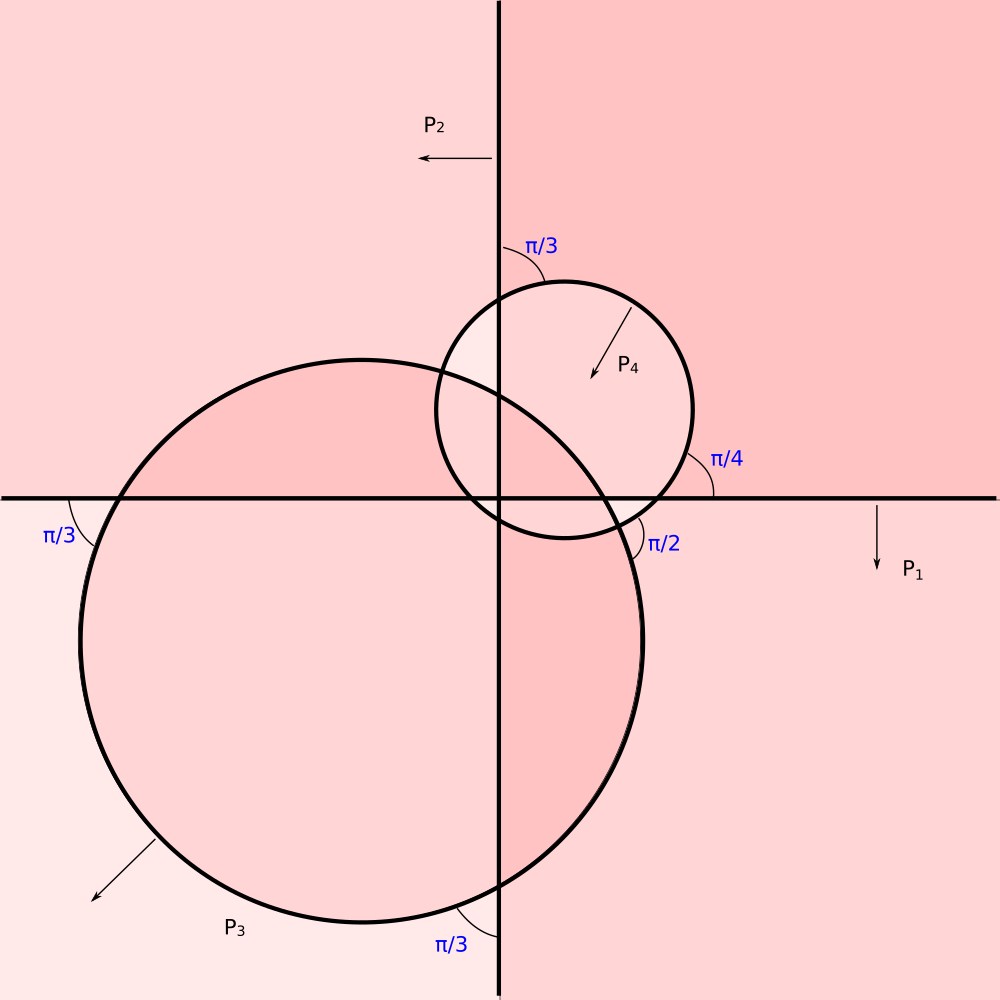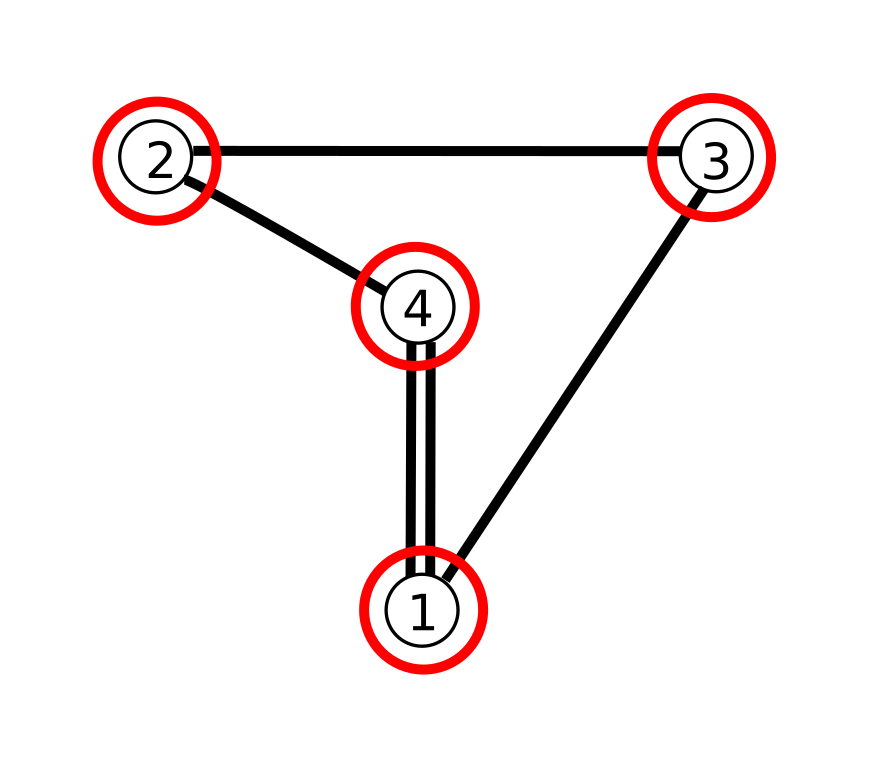Fourth sphere `P_4` with dihedral angles `alpha_(41) = pi/4` `alpha_(42) = pi/3`, `alpha_(43) = pi/2` finishes the hyperbolic tetrahedron.

# Tiling by tetrahedraHere is the tiling.

What is going on?

# Tiling by tetrahedraThis is the tiling.

What is going on?

We are looking in the wrong place!

Plane `z = 0` is the horizon (infinity) of `H^3`.

Tiles become infinitely small at the horizon.

The visible noise is due to finite precision of calculation.

# Tiling in hyperbolic planes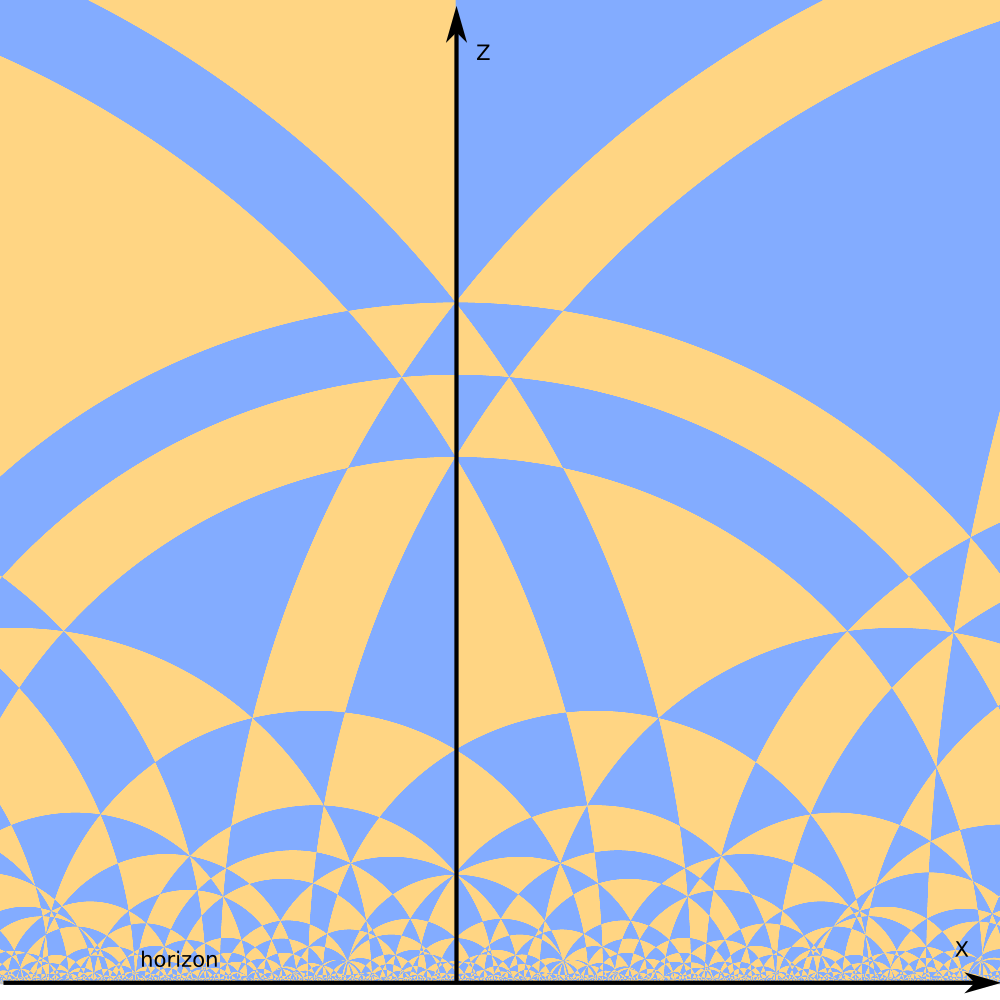"Right" places to look at the space tiling are hyperbolic planes.

One kind of hyperbolic planes in the upper half space model are planes orthogonal to the horizon.

The image looks similar to 2D tiling of hyperbolic plane in the upper half plane model of `H^2`.

However the polygons are not 2D tiles, but odd shaped slices of 3D tiles by hyperbolic plane.

# Tiling in hyperbolic planes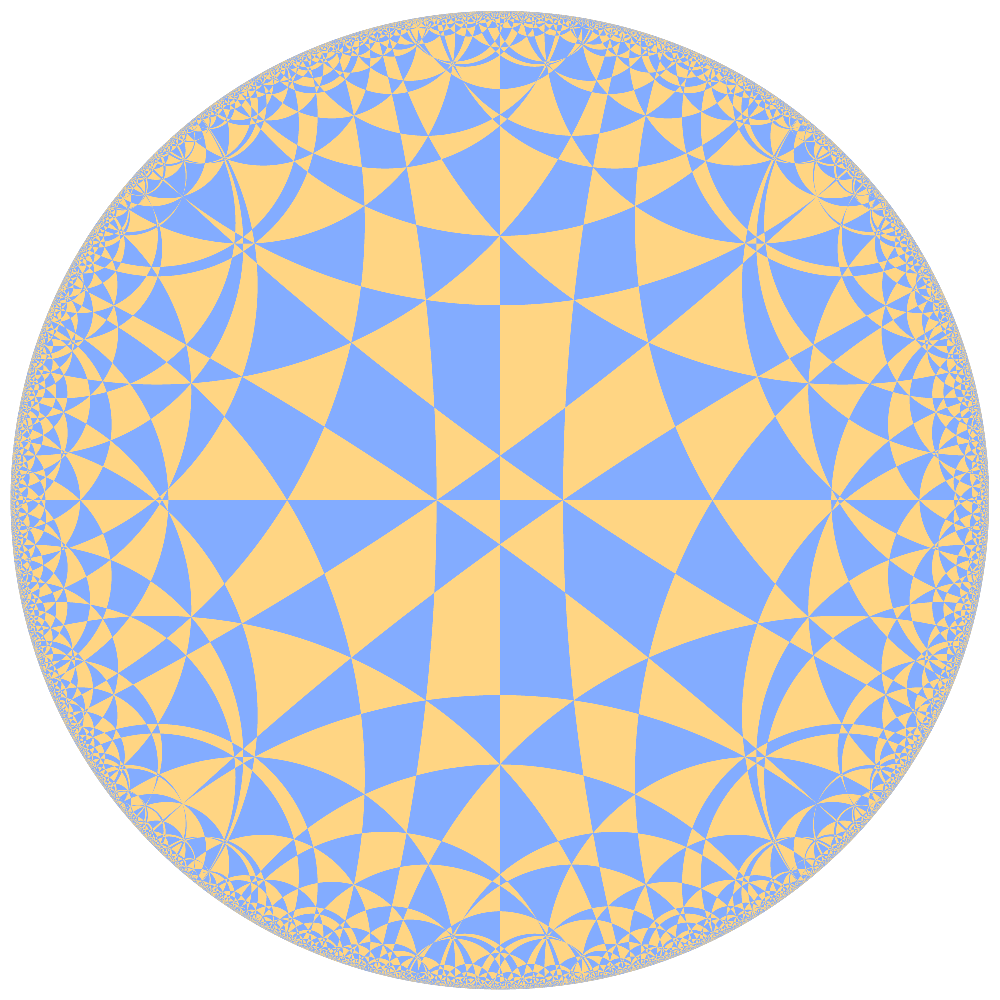Another type of hyperbolic planes are half spheres centered at the horizon (image below). The planes can be flattened by stereographic projection.

The flattened tiling (on the left) looks similar to the 2D tilings in the Poincare circle model of `H^2`.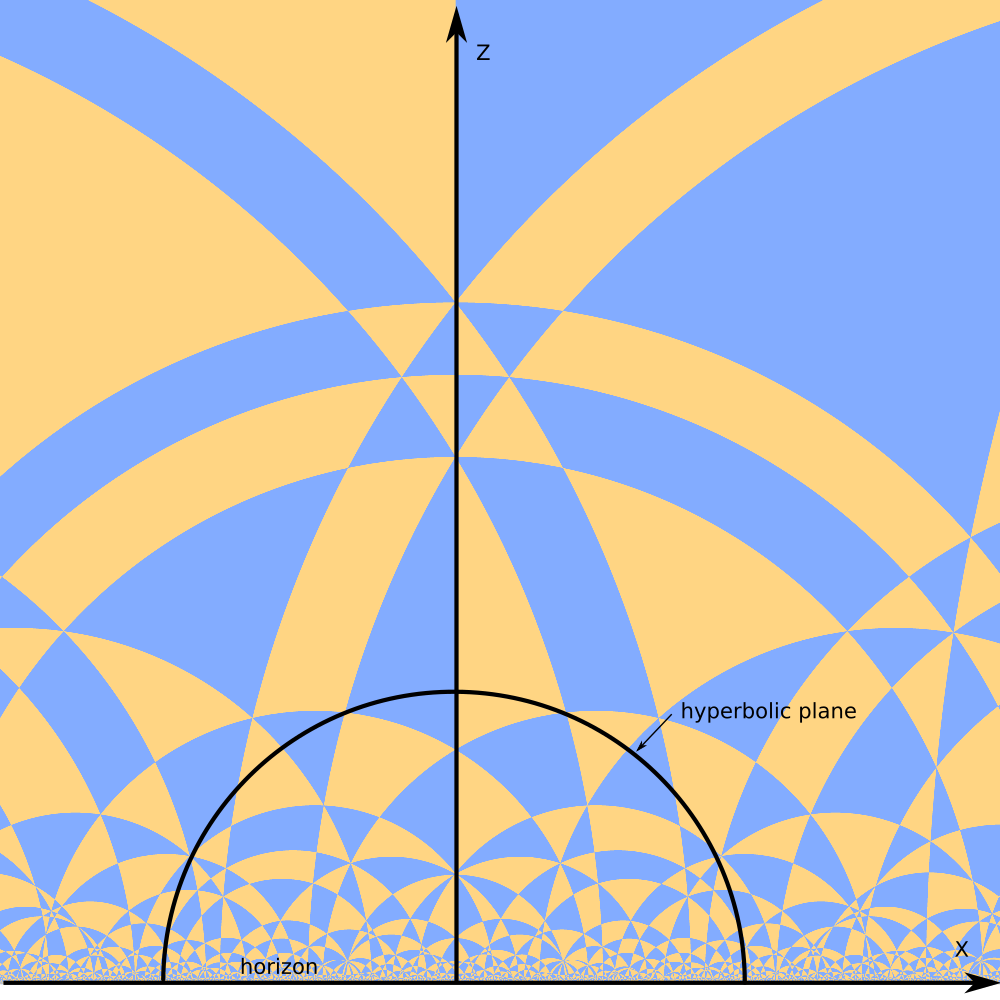# "Problems" of hyperbolic tilings

The main feature of hyperbolic tilings visualization is high range of visual scale.

In the circle model of `H^2` we have few regular sized tiles near the center. Tiles shrink rapidly as we approach the horizon.

Upper half plane model `H^2` has similar behavior. Tiles shrink rapidly as we approach the horizon and stretch rapidly as we move up.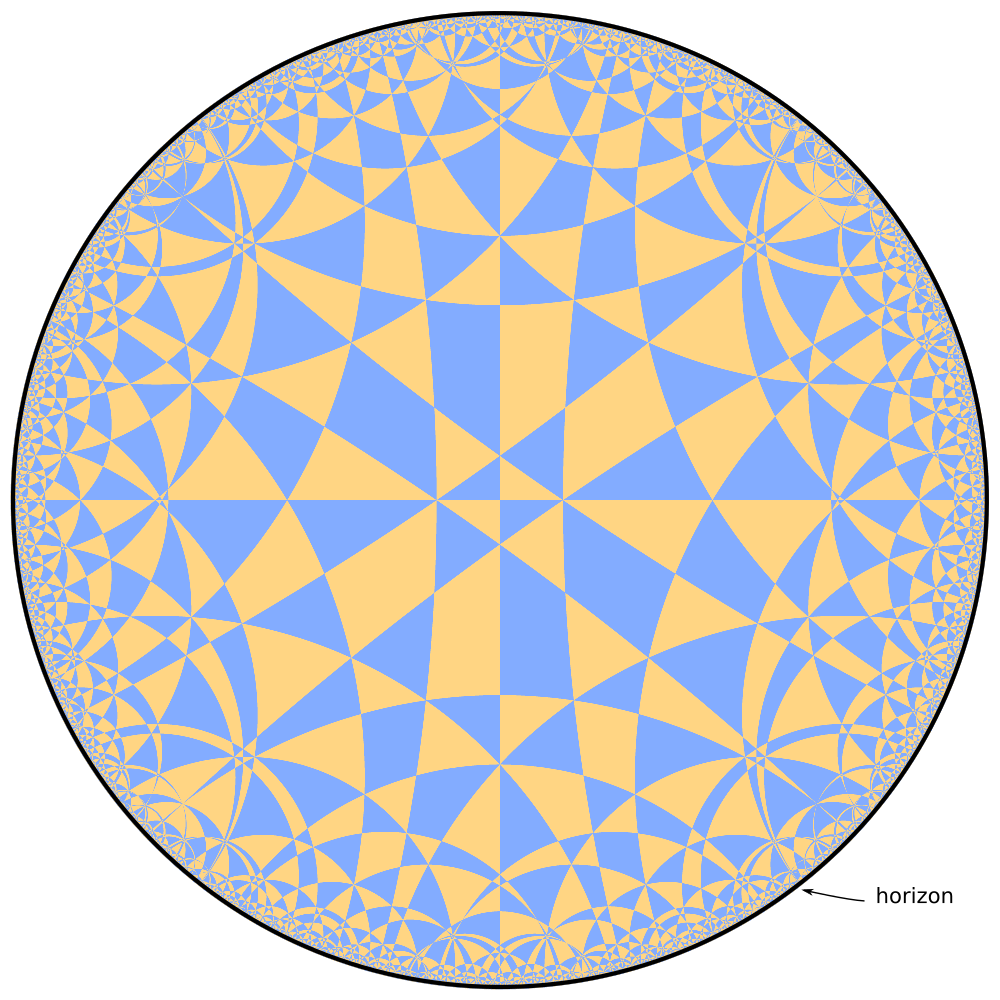# Introducing horospheres

Let's "flatten" the hyperbolic plane.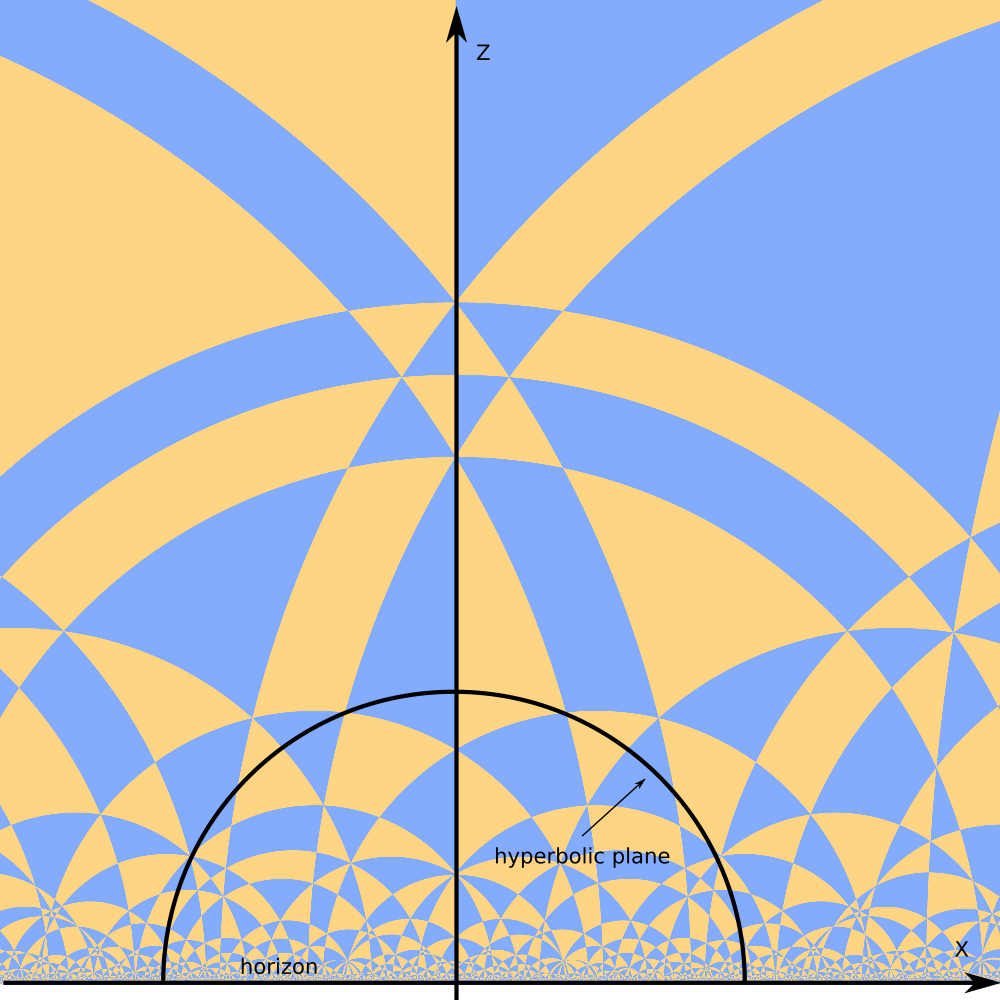# Horosphere (2)

Let's "flatten" the hyperbolic plane.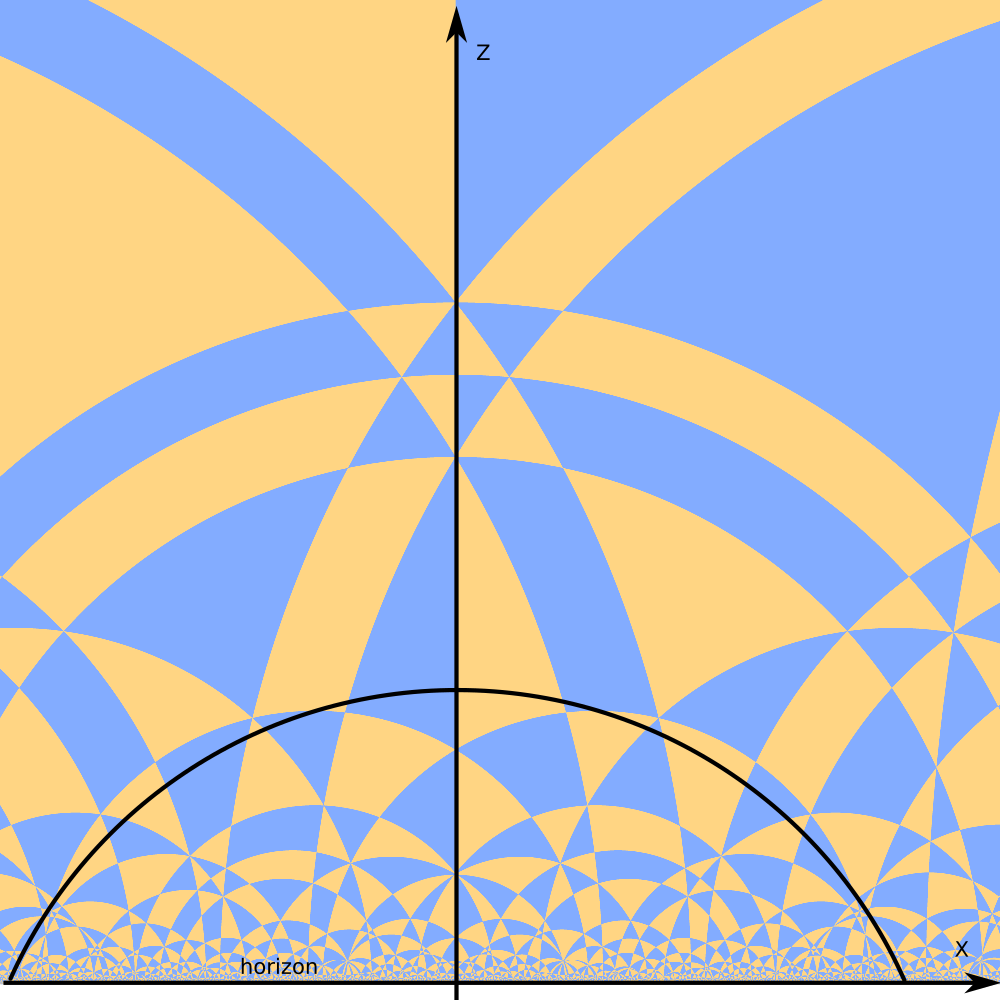# Horosphere (3)

Let's "flatten" the hyperbolic plane.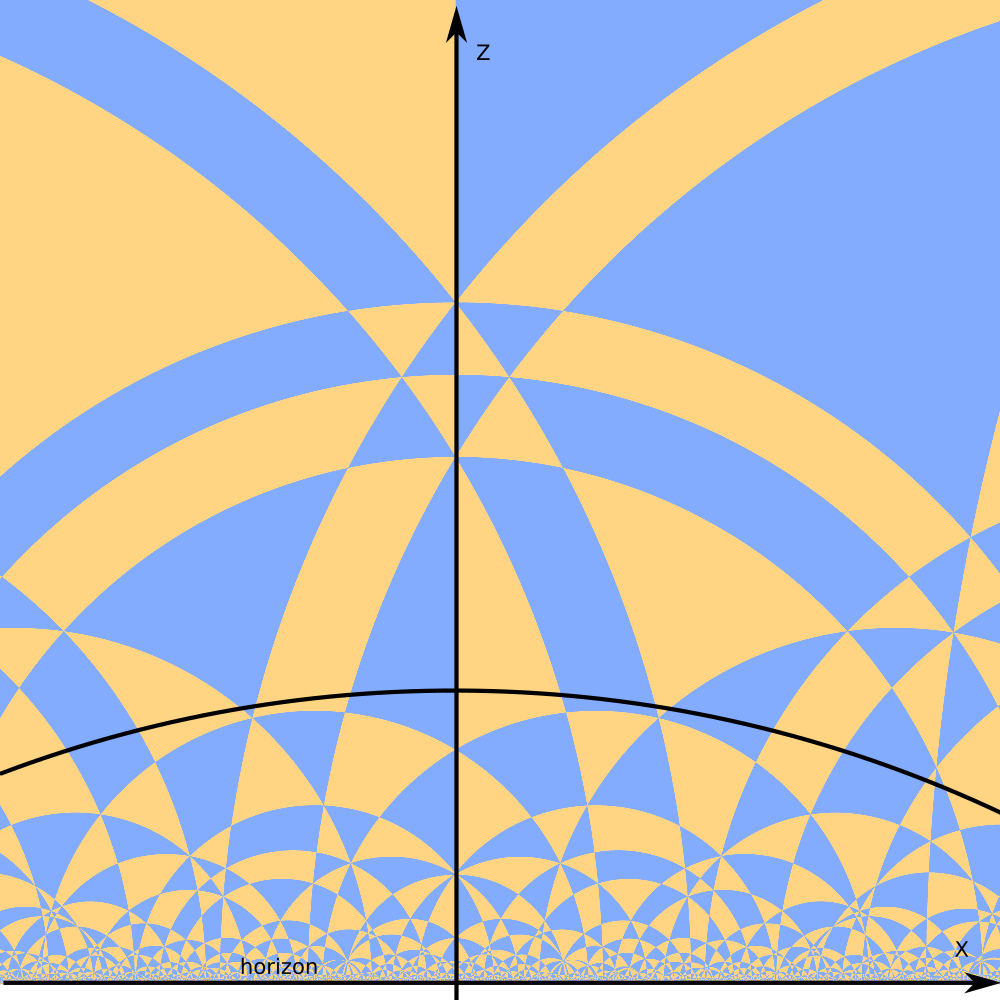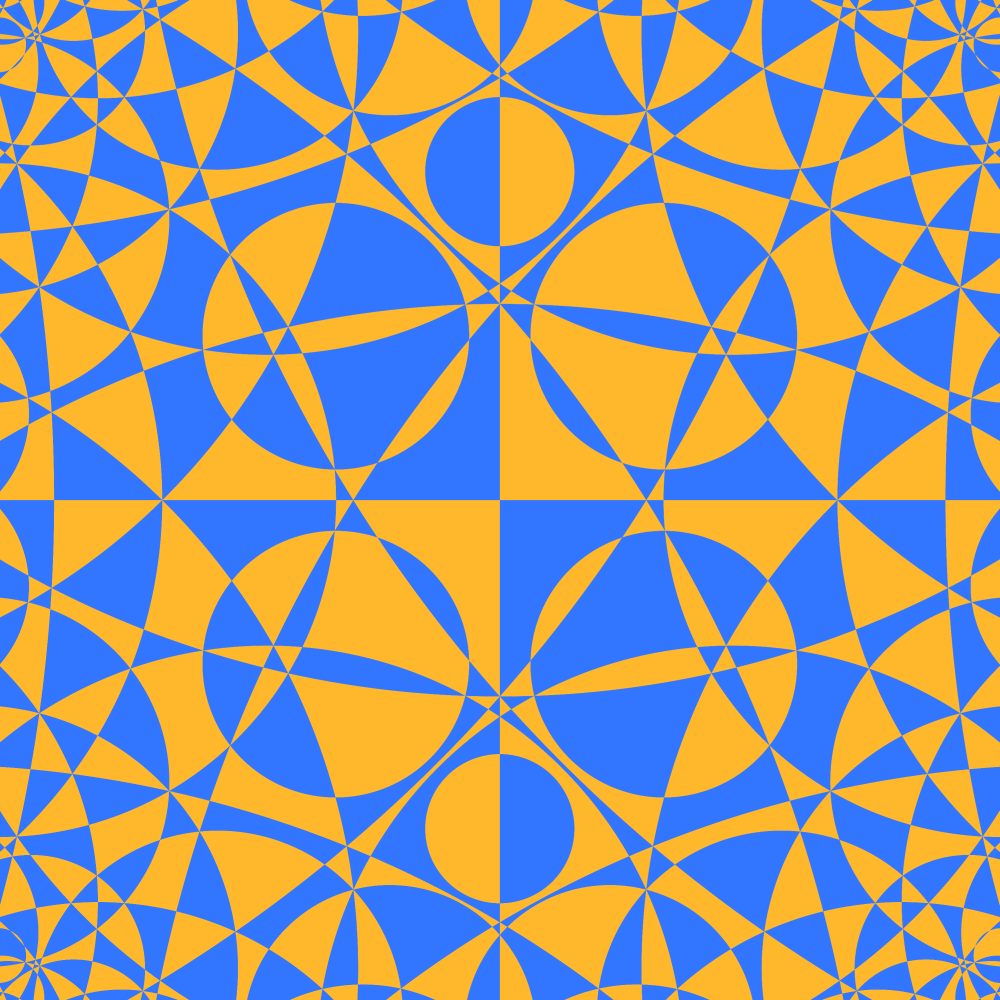# Horosphere (4)

We got horosphere.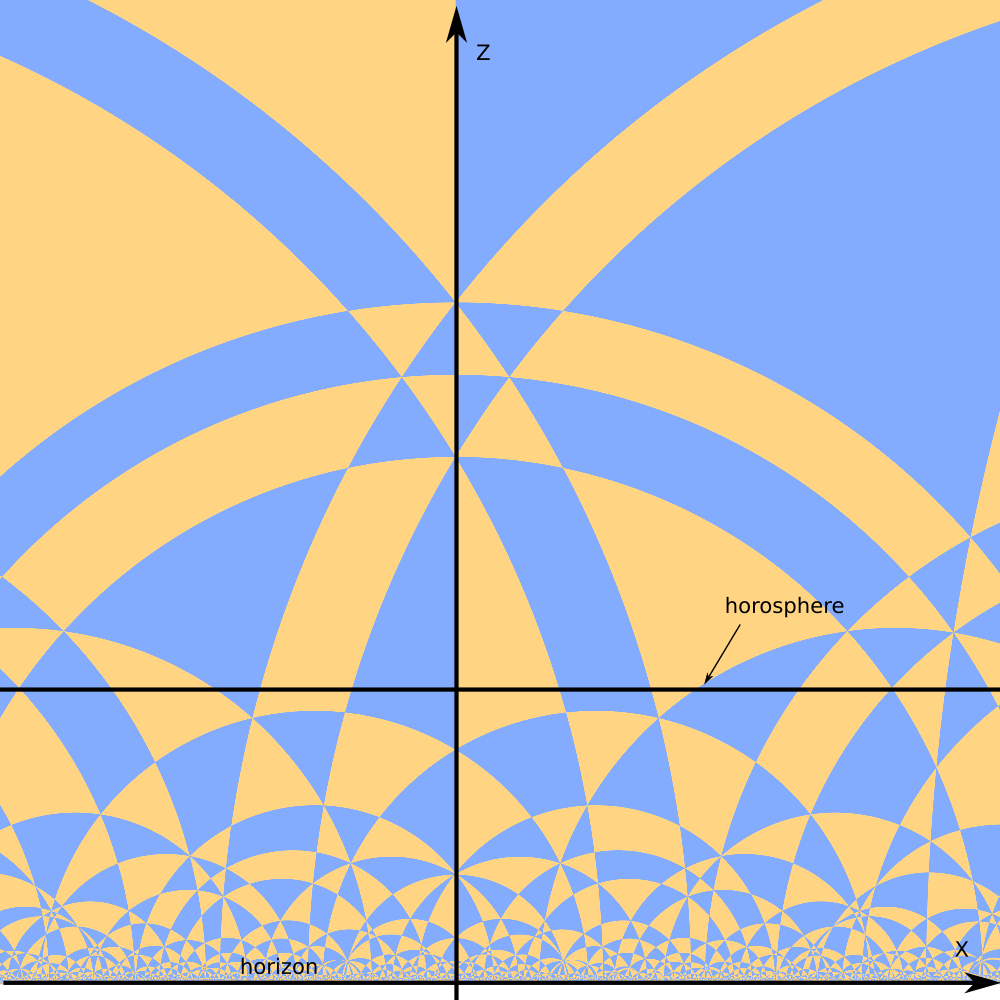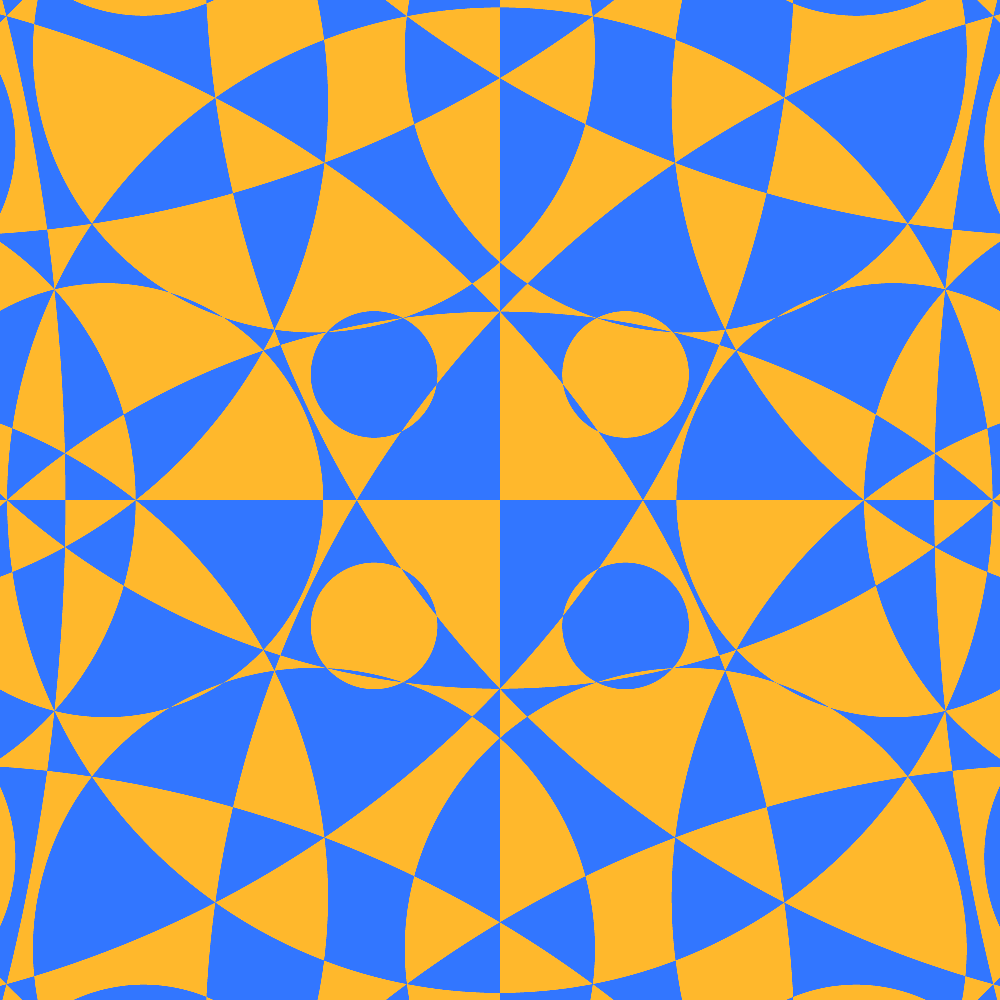# Horosphere (5)

Zooming out the camera.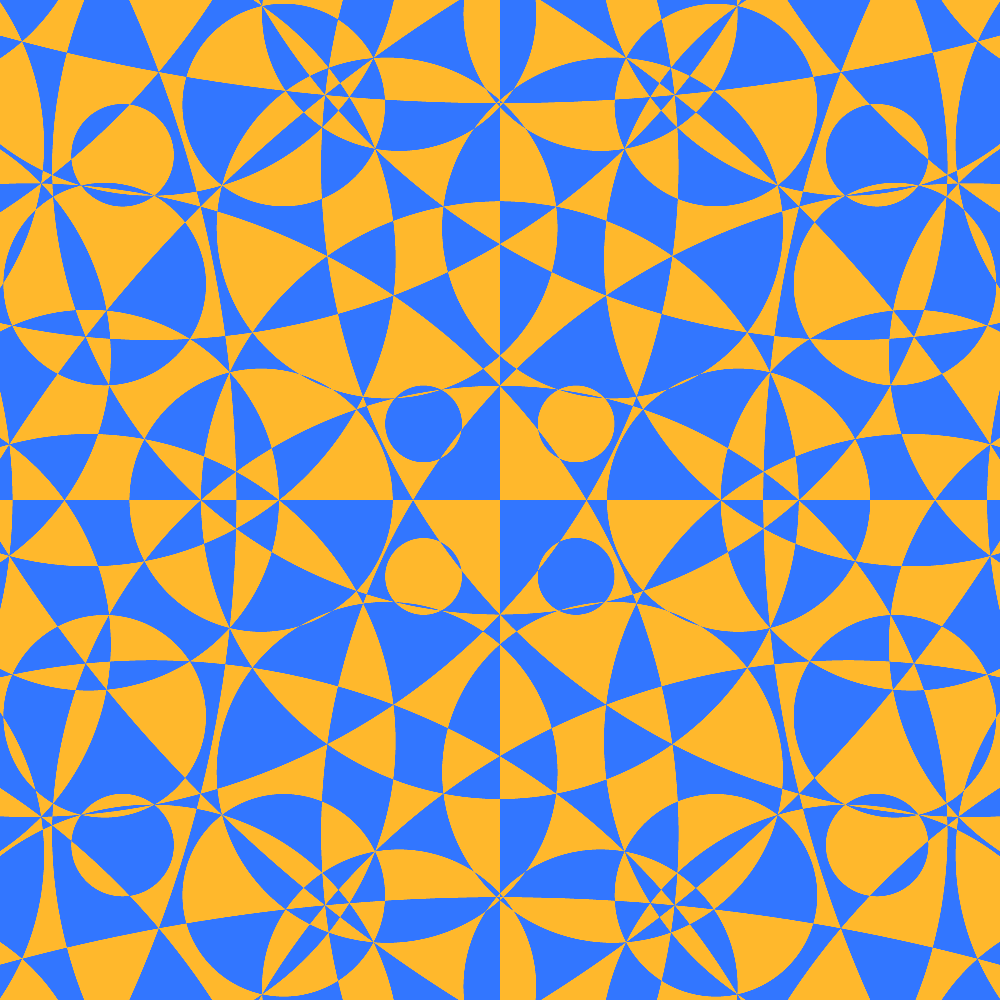# Horosphere (6)

Zooming out the camera.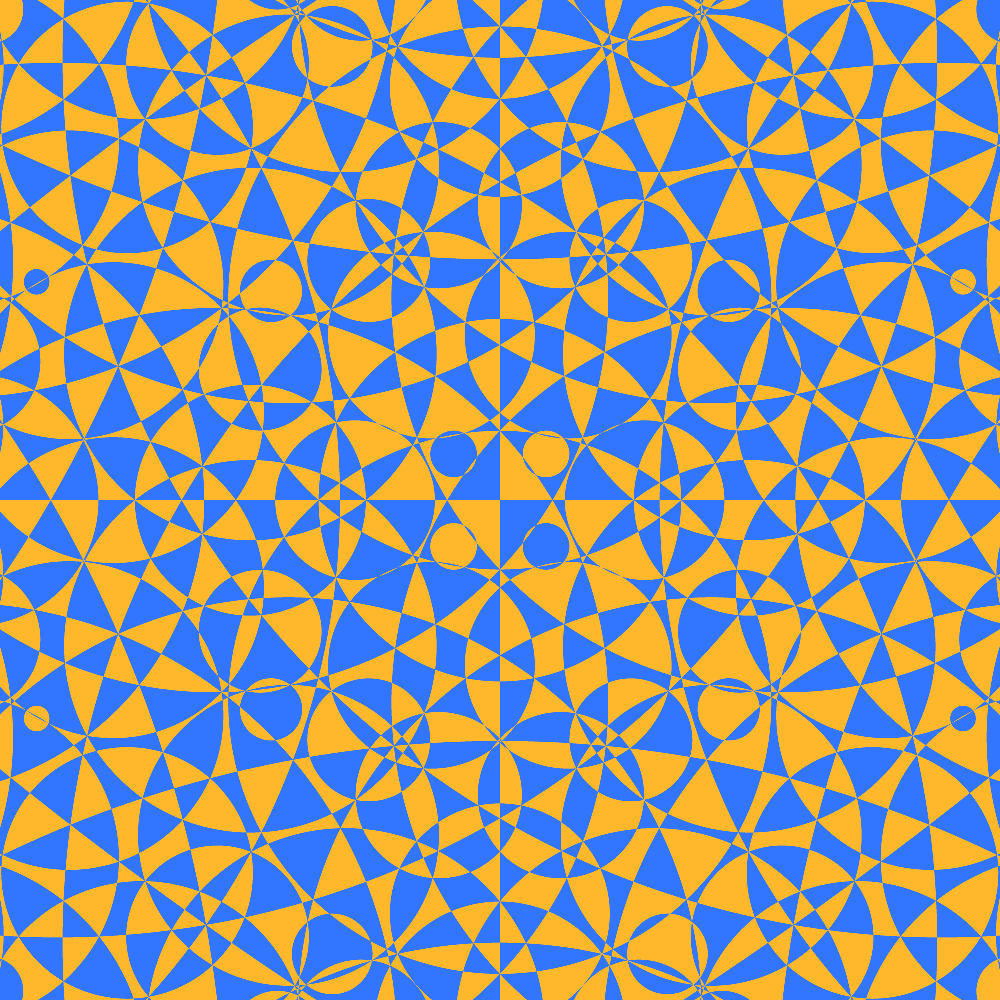# Horosphere (7)

Zooming out the camera.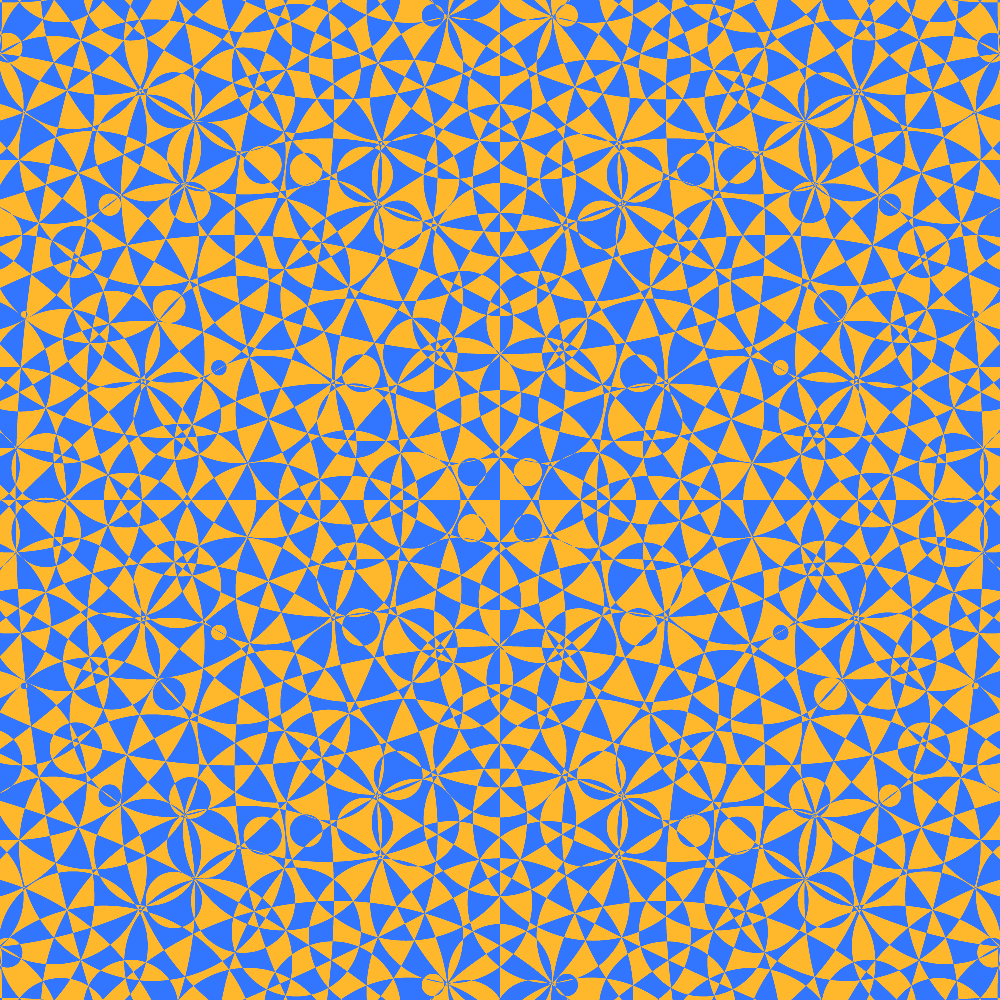# Horosphere (8)

Zooming out the camera. Animaton https://youtu.be/u1Lp9NS98E0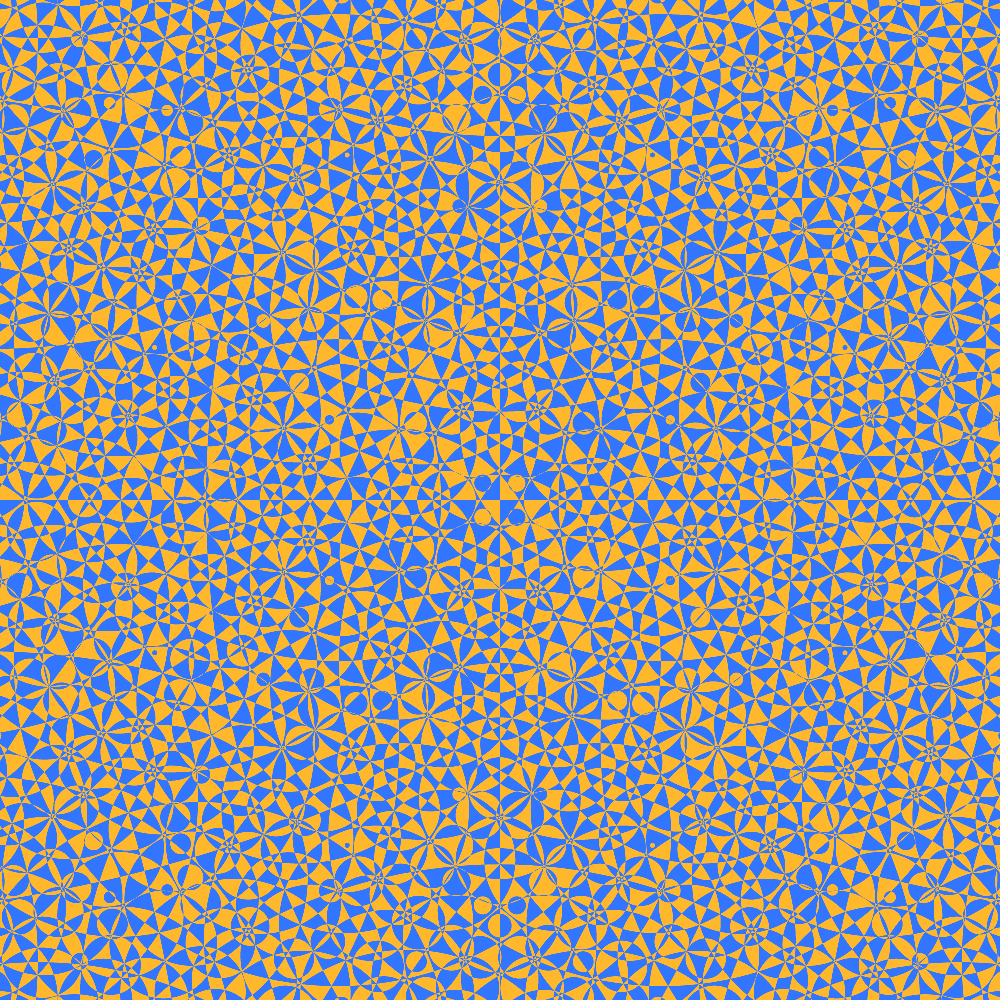# Horosphere Motion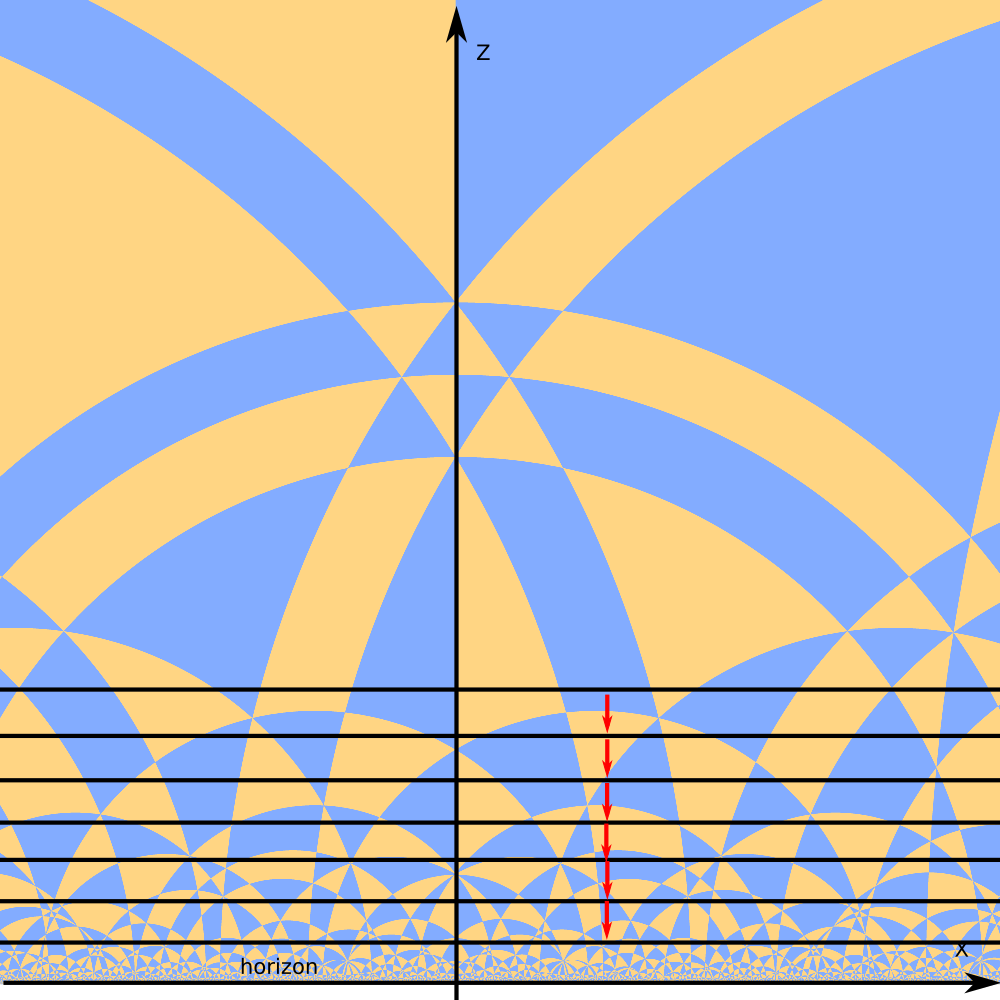There are different motion we can perform with horospere

Straight hyperbolic translation. Animaton
https://youtu.be/PKauqA_Qp9Q

# Horosphere Motion 2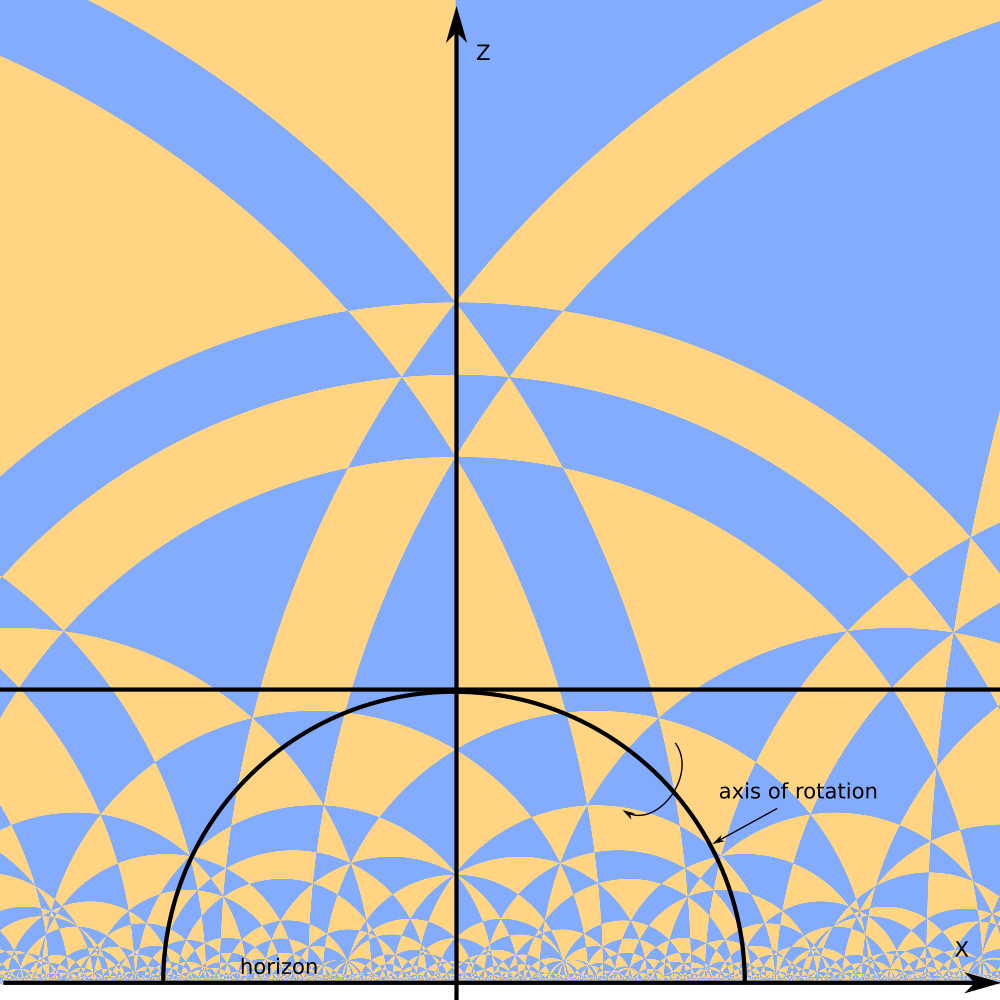There are different motion we can perform with horospere

Hyperbolic rotation around hyperbolic straight line.
Animaton 1.
https://youtu.be/PhcbmsTYc-0
animaton 2.
https://youtu.be/rSEzGtp56vI

# Horosphere Motion 3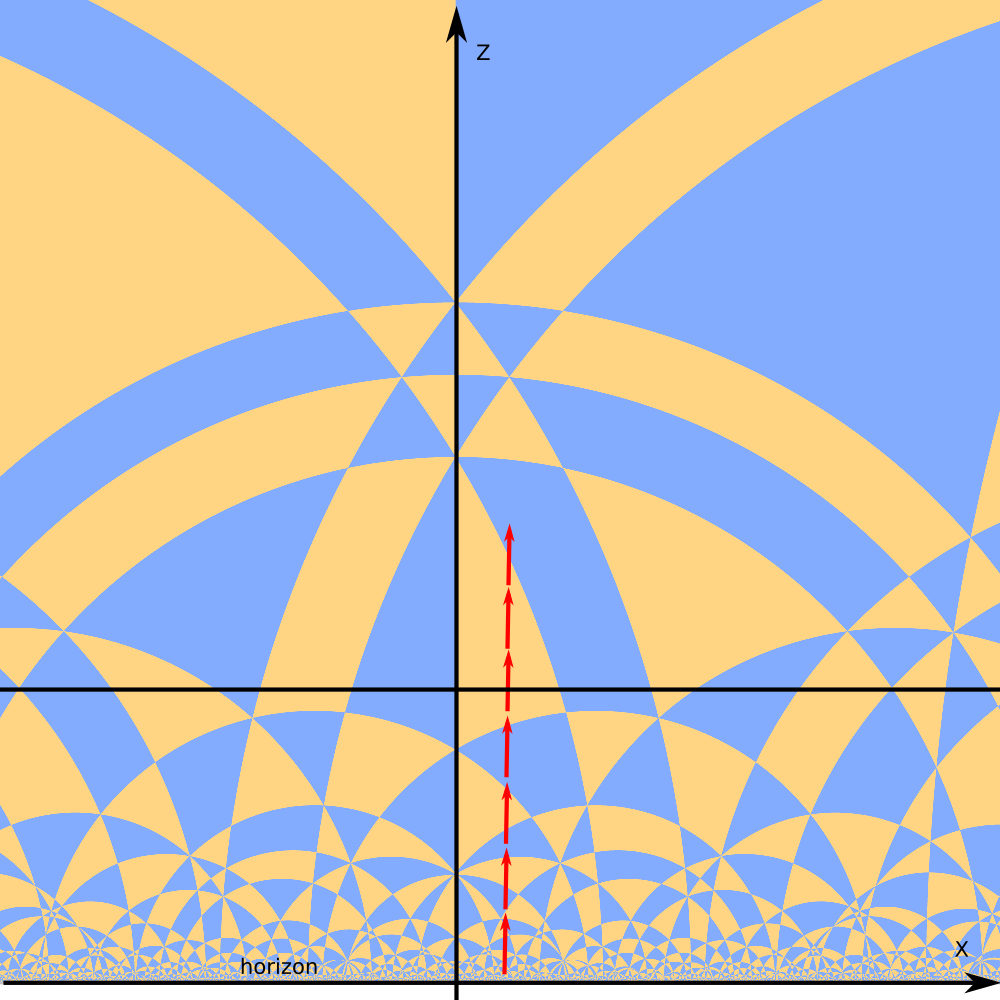There are different motion we can perform with horospere

Moving tiling past horosphere.

Similar to horosphere hyperbolic translation.

The difference is that scale of the tiling remain the same.

Animation.
https://youtu.be/gGvlkomzvAg

# Different Hyperbolic Tetrahedra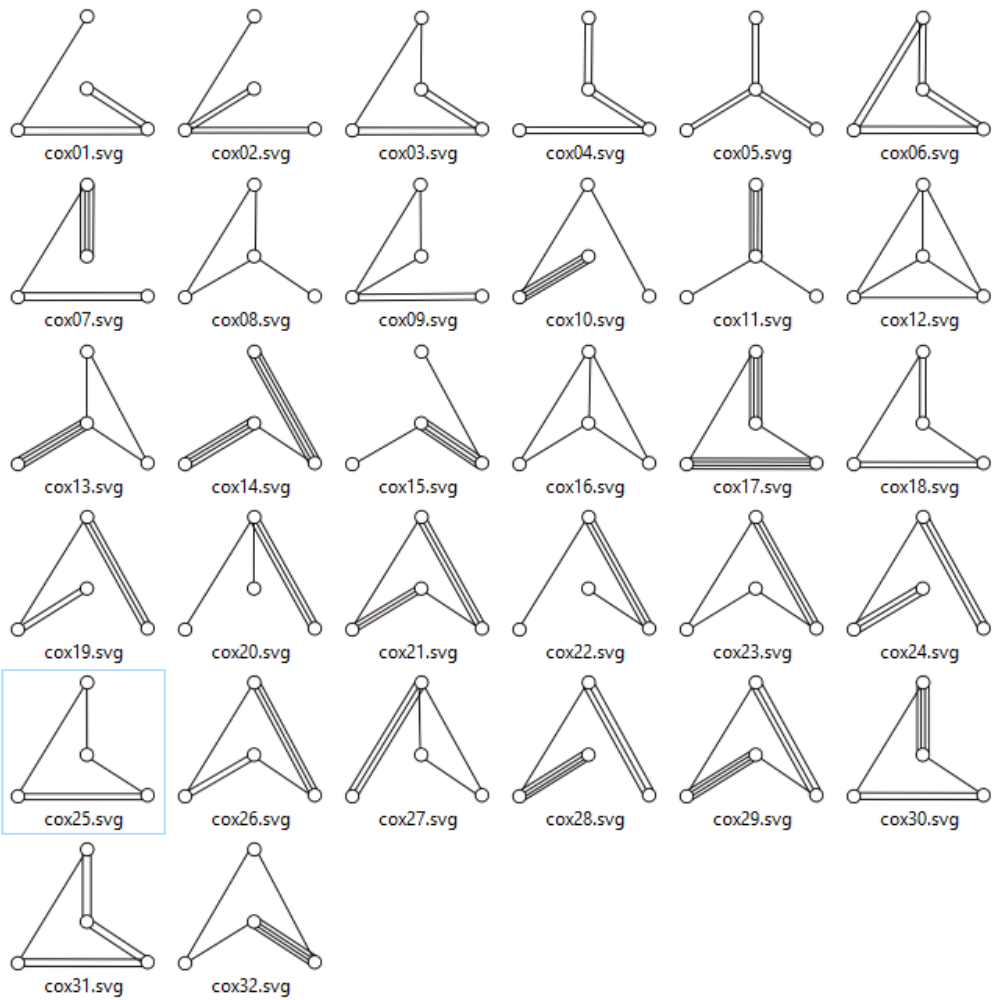There are 32 compact hyperbolic tetrahedra with kaleidoscopic angles.

There is infinite number of non compact tetrahedra (tetrahedra with vertices beyond infinity).

There is infinite number of more complex polyhedra.

A lot of possibilities for fun.

# Tetrahedron example 1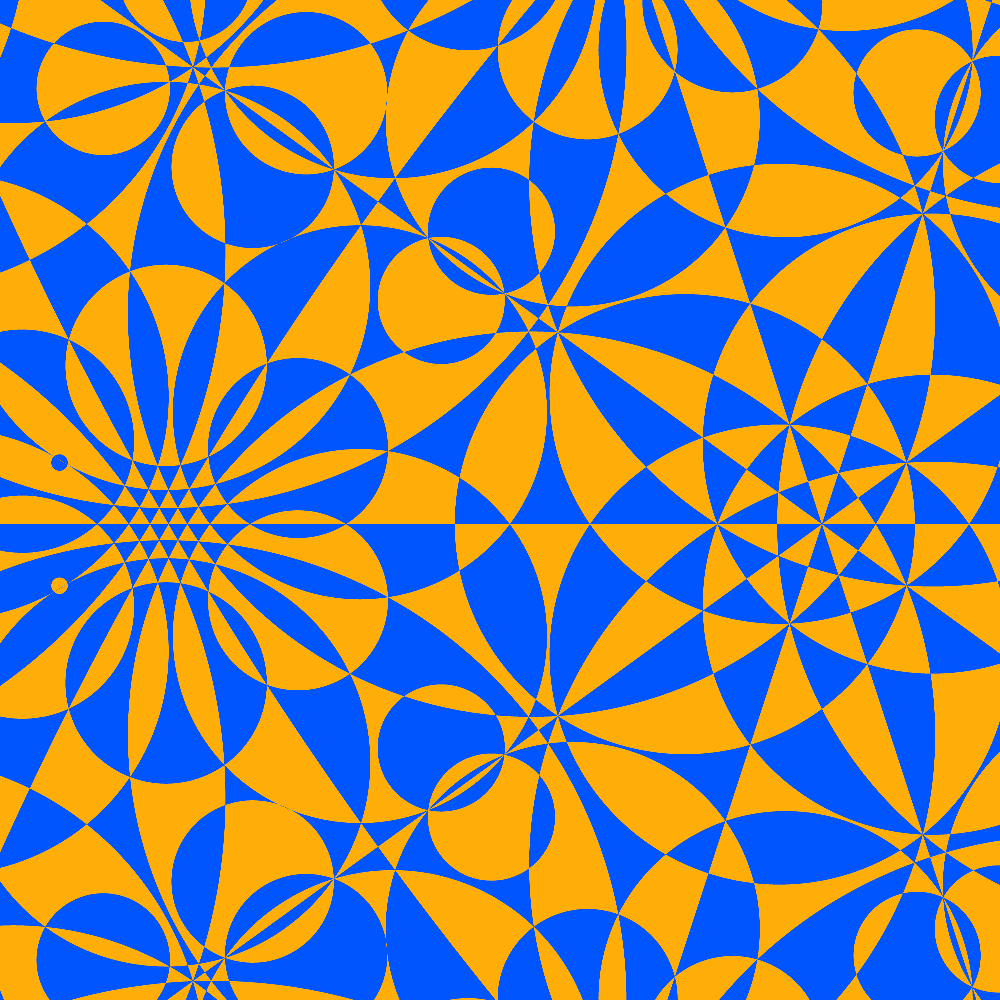This tetrahedron has icosahedral vertex `**532` and ideal vertex `**333`.

Animation.
https://youtu.be/AVnNs3dU3-c

# Tetrahedron example 2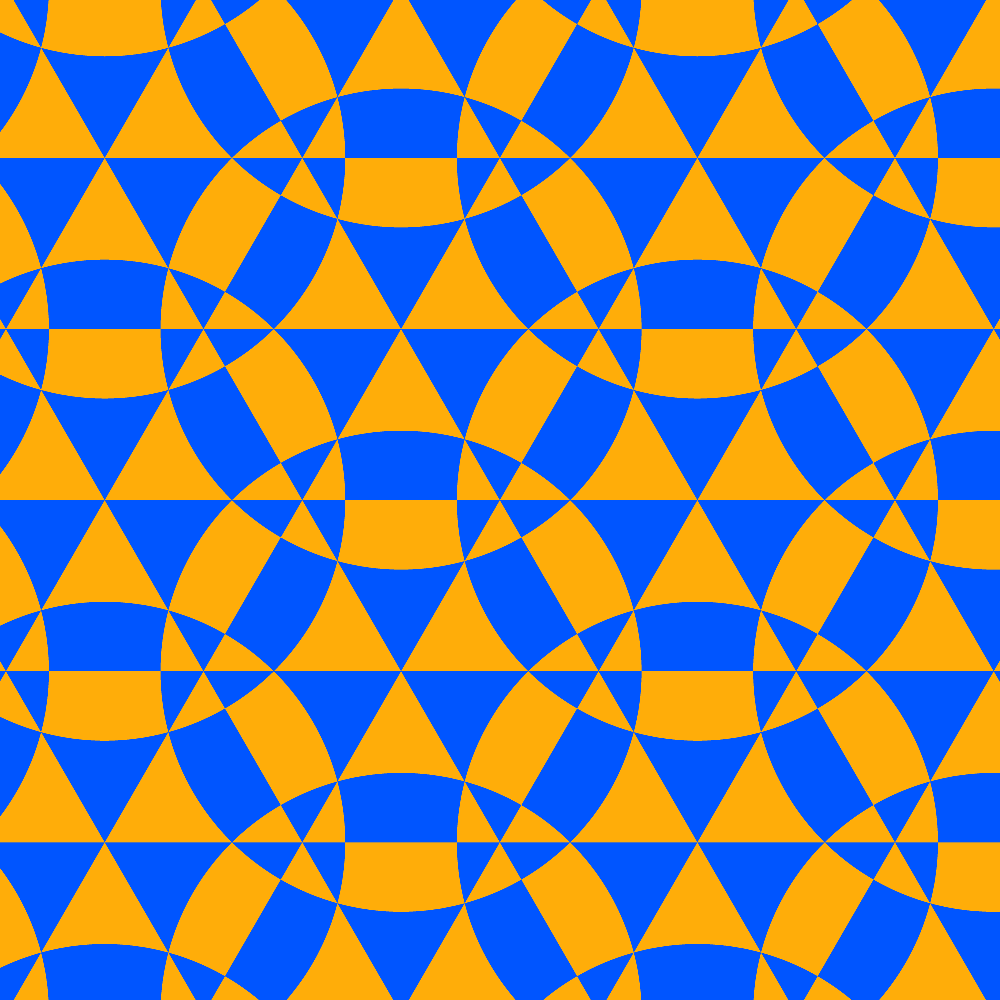Tetrahedron has ideal vertex `**333`.

Horosphere is moving away from that vertex.

Animation.
https://youtu.be/F1cDHtS6oeE

# Other Cross Sections

Slicing euclidean tiling wih plane also produces interesting results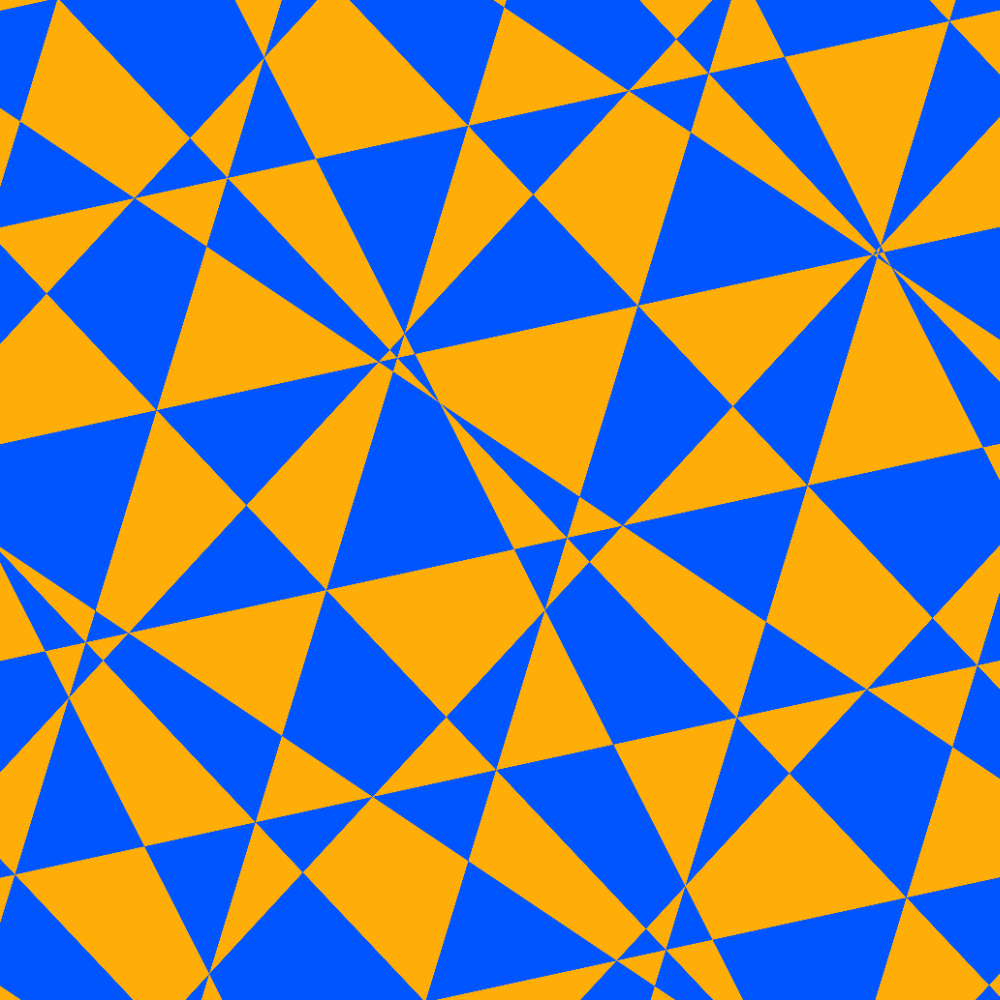Animation 1
https://youtu.be/tR_IFchXvo8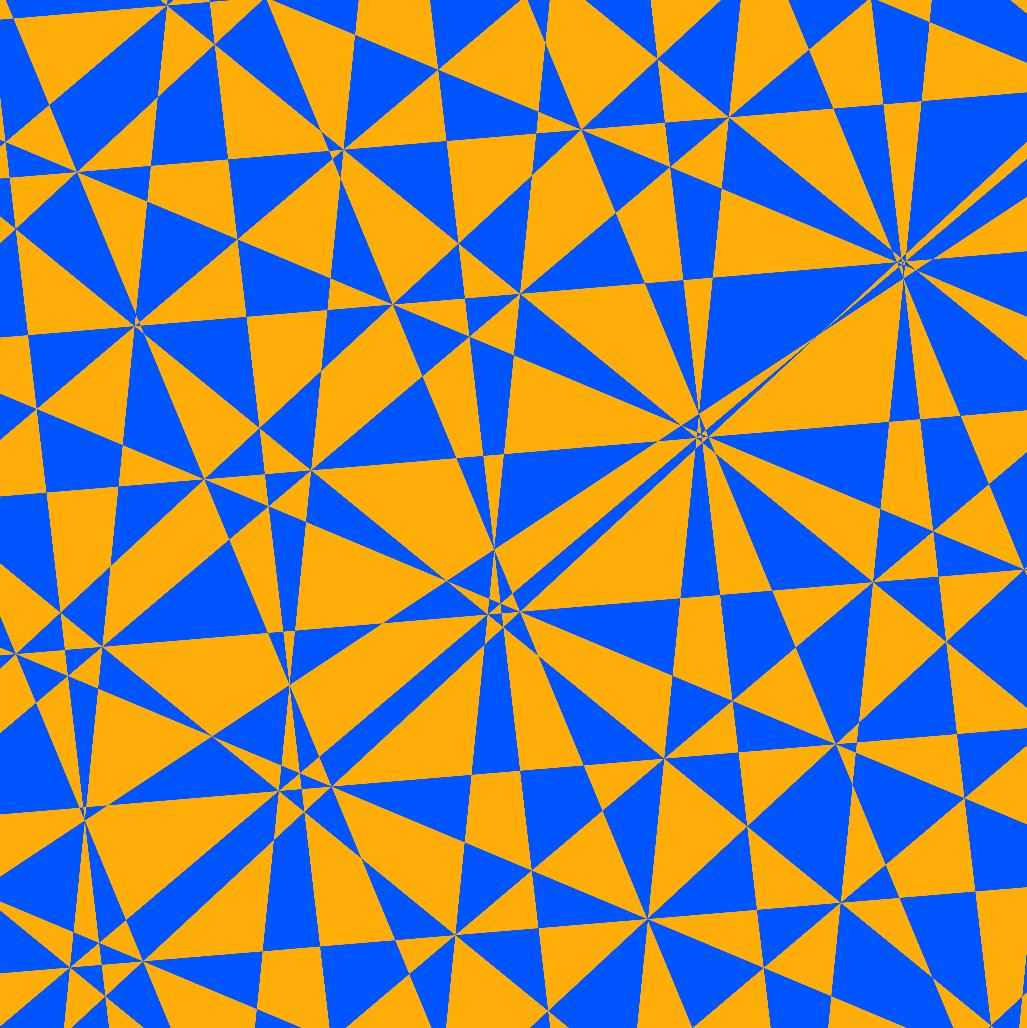Animation 2
https://youtu.be/-vzEcbNrFnk

# Spherical Cross Sections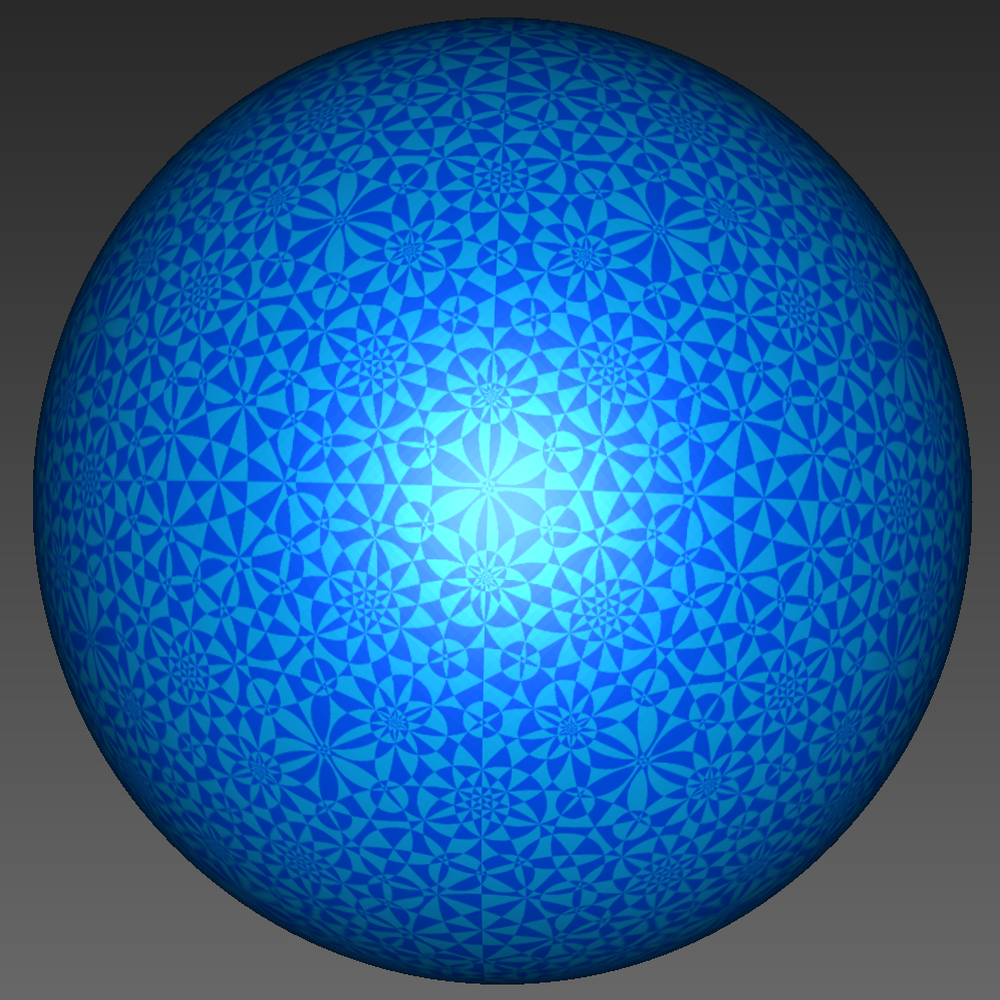VRML model.

# 3D Printing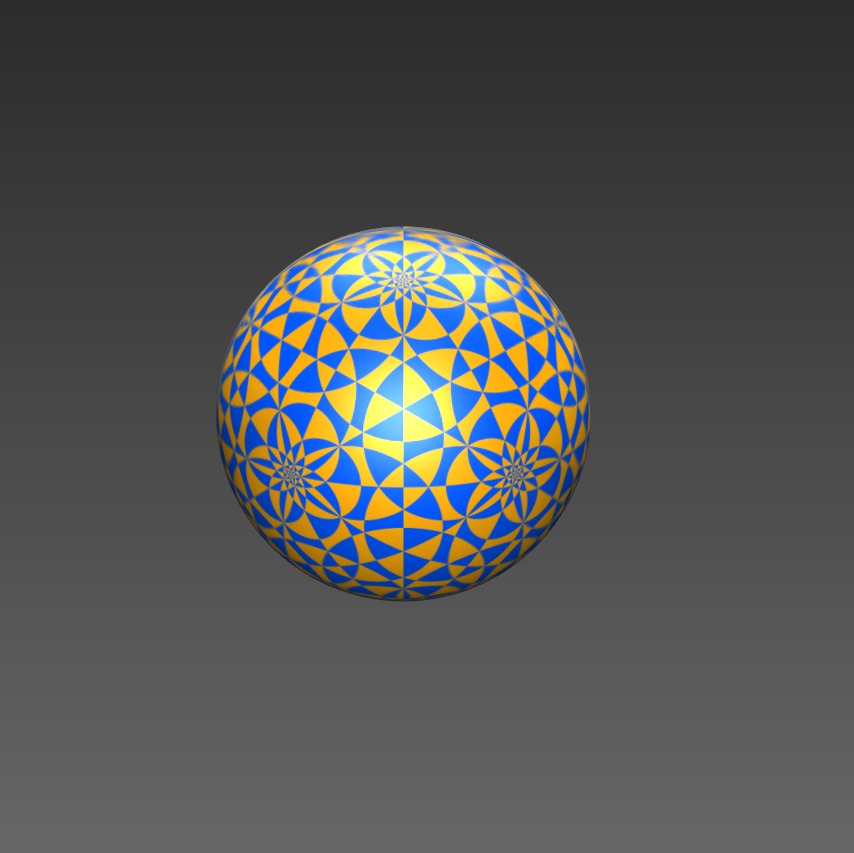3D printing involves creation of spherical color map and applying it to a shape (sphere).

The modeling was done in ShapeJS - online tool for making 3d printable models via script.

The 3D printing was done by Shapeways.# Real Time Rendering with WebGL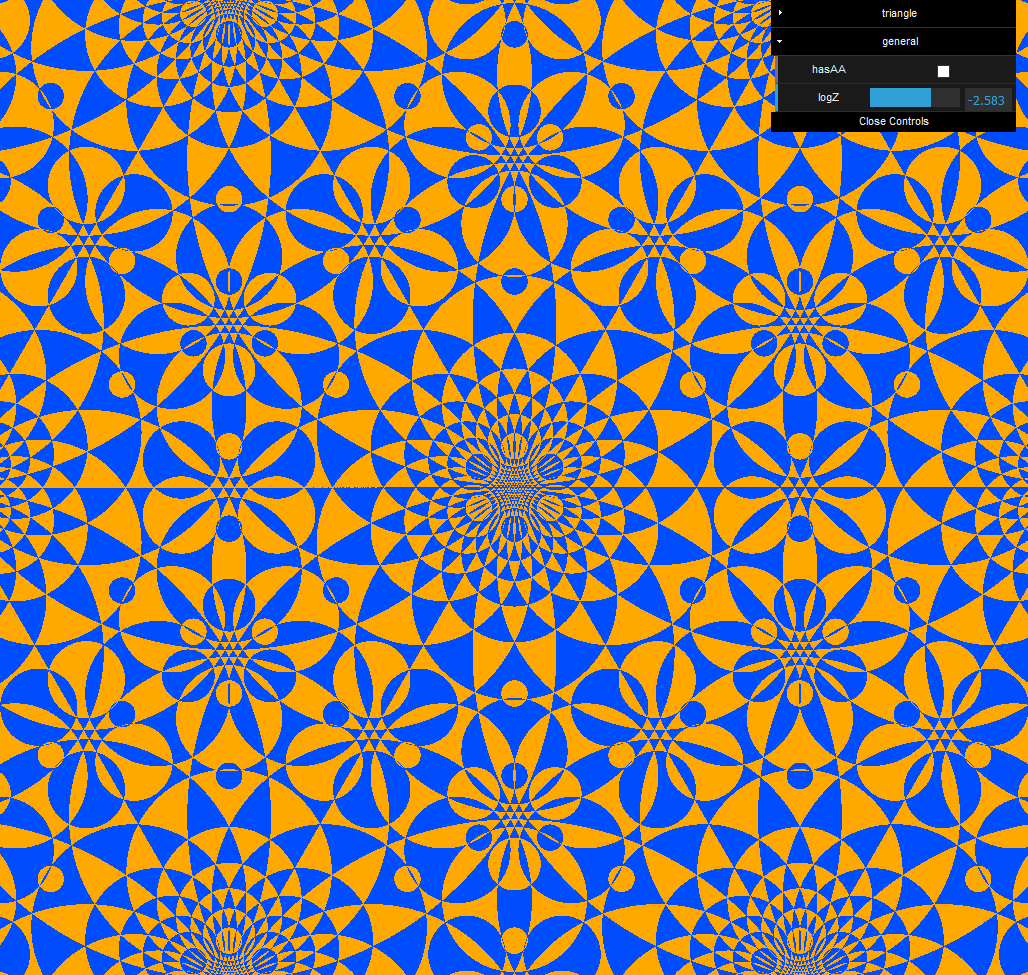WebGL is web browser API which allow web pages run graphics program directly on users GPU (graphics card).

# End